GetFEM  5.4.2
getfem Namespace Reference

GEneric Tool for Finite Element Methods. More...

## Classes

class  abstract_constraints_projection
Abstract projection of a stress tensor onto a set of admissible stress tensors. More...

class  abstract_hyperelastic_law
Base class for material law. More...

struct  abstract_xy_function
below a list of simple functions of (x,y) used for building the crack singular functions More...

class  accumulated_distro
Takes a matrix or vector, or vector of matrices or vectors and creates an empty copy on each thread. More...

struct  Ciarlet_Geymonat_hyperelastic_law
Ciarlet-Geymonat hyperelastic law. More...

class  context_dependencies
Deal with interdependencies of objects. More...

class  copyable_ptr
A wrapper around a unique_ptr that clones the value on copy. More...

class  dx_export
A (quite large) class for exportation of data to IBM OpenDX. More...

class  fem
virtual_fem implementation as a vector of generic functions. More...

class  fem_global_function
fem object with global basis functions. More...

class  fem_interpolation_context
structure passed as the argument of fem interpolation functions. More...

class  fem_level_set
FEM associated with getfem::mesh_fem_level_set objects. More...

class  fem_precomp_
Pre-computations on a fem (given a fixed set of points on the reference convex, this object computes the value/gradient/hessian of all base functions on this set of points and stores them. More...

class  fem_precomp_pool
handle a pool (i.e. More...

struct  generalized_Blatz_Ko_hyperelastic_law
Blatz_Ko hyperelastic law. More...

class  generic_assembly
Generic assembly of vectors, matrices. More...

class  global_function
inherit from this class to define new global functions. More...

Thread policy, regulated by partition_master (can be true thread- or partition-based) More...

class  im_data
im_data provides indexing to the integration points of a mesh im object. More...

class  integration_method
this structure is not intended to be used directly. More...

class  interpolated_fem
FEM which interpolates a mesh_fem on a different mesh. More...

struct  interpolator_on_mesh_fem
a general structure for interpolation of a function defined by a mesh_fem and a vector U at any point (interpolation of value and gradient). More...

class  level_set
Define a level-set. More...

class  mat_elem_type
Description of an elementary matrix. More...

struct  membrane_elastic_law
Linear law for a membrane (plane stress), orthotropic material caracterized by Ex, Ey, vYX and G, with optional orthotropic prestresses. More...

class  mesh
Describe a mesh (collection of convexes (elements) and points). More...

class  mesh_fem
Describe a finite element method linked to a mesh. More...

class  mesh_fem_global_function
this is a convenience class for defining a mesh_fem with base functions which are global functions (functions defined across more than one convexes of a mesh) given by the user. More...

class  mesh_fem_sum
Implement a special mesh_fem with merges the FEMs of two (or more) mesh_fems. More...

class  mesh_im
Describe an integration method linked to a mesh. More...

class  mesh_im_cross_level_set
Describe an adaptable integration method linked to a mesh cut by at least two level sets on the intersection of two level sets. More...

class  mesh_im_level_set
Describe an adaptable integration method linked to a mesh cut by a level set. More...

class  mesh_level_set
Keep informations about a mesh crossed by level-sets. More...

class  mesh_region
structure used to hold a set of convexes and/or convex faces. More...

class  mesh_slice_cv_dof_data
Use this structure to specify that the mesh must be deformed before the slicing operation (with a mesh_fem and an associated field). More...

class  mesh_slicer
Apply a serie a slicing operations to a mesh. More...

class  model
‘Model’' variables store the variables, the data and the description of a model. More...

struct  Mooney_Rivlin_hyperelastic_law
Mooney-Rivlin hyperelastic law. More...

struct  Neo_Hookean_hyperelastic_law
Neo-Hookean hyperelastic law variants. More...

class  nonlinear_elem_term
abstract class for integration of non-linear terms into the mat_elem computations the nonlinear term is added into the mat_elem_type via mat_elem_nonlinear More...

class  omp_distribute
Use this template class for any object you want to distribute to open_MP threads. More...

class  parallel_boilerplate
Encapsulates open_mp-related initialization and de-initialization. More...

class  partial_mesh_fem
a subclass of mesh_fem which allows to eliminate a number of dof of the original mesh_fem. More...

class  partition_iterator
Iterator that runs over partitions on the current thread and sets the global (but thread-specific) partition during incrementation. More...

class  partition_master
A singleton that Manages partitions on individual threads. More...

struct  plane_strain_hyperelastic_law
Plane strain hyperelastic law (takes another law as a parameter) More...

class  poly_integration
Description of an exact integration of polynomials. More...

class  pos_export
POS export. More...

class  projected_fem
FEM which interpolates the projection of a mesh_fem on a different mesh. More...

struct  SaintVenant_Kirchhoff_hyperelastic_law
Saint-Venant Kirchhoff hyperelastic law. More...

class  slicer_action
generic slicer class. More...

class  slicer_boundary
Extraction of the boundary of a slice. More...

class  slicer_build_edges_mesh
Slicer whose side-effect is to build the list of edges (i.e. More...

class  slicer_build_mesh
Slicer whose side-effect is to build a mesh from the slice simplexes. More...

class  slicer_build_stored_mesh_slice
a getfem::mesh_slicer whose side effect is to build a stored_mesh_slice object. More...

class  slicer_complementary
Build the complementary of a slice. More...

class  slicer_compute_area
Slicer whose side-effect is to compute the area of the slice. More...

class  slicer_cylinder
Slices a mesh with a cylinder (or its boundary). More...

class  slicer_explode
Contract or expand each convex with respect to its gravity center. More...

class  slicer_half_space
Slice a mesh with a half-space (or its boundary). More...

class  slicer_intersect
Build the intersection of two slices. More...

class  slicer_isovalues
Extract an isosurface. More...

class  slicer_mesh_with_mesh
Slices a mesh with another mesh. More...

class  slicer_none
This slicer does nothing. More...

class  slicer_sphere
Slices a mesh with a sphere (or its boundary). More...

class  slicer_union
union of two slices More...

class  slicer_volume
Base class for general slices of a mesh (planar, sphere, cylinder,isosurface) More...

class  standard_locale
Identical to gmm::standard_locale, but does not change std::locale in multi-threaded sections of the code, which is not thread-safe. More...

class  stored_mesh_slice
The output of a getfem::mesh_slicer which has been recorded. More...

class  temporary_mesh_deformator
An object function that first deforms and then remembers to restore a mesh if it has to be restored for other bricks. More...

Allows to re-throw exceptions, generated in OpemMP parallel section. More...

class  torus_fem

class  torus_mesh
Copy an original 2D mesh to become a torus mesh with radial dimension. More...

class  torus_mesh_fem
Mesh fem object that adapts. More...

class  virtual_brick
The virtual brick has to be derived to describe real model bricks. More...

class  virtual_dispatcher
The time dispatcher object modify the result of a brick in order to apply a time integration scheme. More...

class  virtual_fem
Base class for finite element description. More...

class  virtual_time_scheme
The time integration scheme object provides the necessary methods for the model object to apply a time integration scheme to an evolutionnary problem. More...

class  VM_projection
Von Mises projection. More...

class  vtk_export
VTK/VTU export. More...

## Typedefs

typedef dof_description * pdof_description
Type representing a pointer on a dof_description.

typedef std::shared_ptr< const getfem::virtual_fempfem
type of pointer on a fem description More...

typedef std::shared_ptr< const virtual_brickpbrick
type of pointer on a brick

## Enumerations

enum  integration_method_type
the list of main integration method types

## Functions

template<class T >
void gen_add (const T &a, T &b)
Generic addition for gmm types as well as vectors of gmm types.

template<class T >
void equal_resize (T &a, const T &b)
Resize 'a' to the same size as 'b'. More...

template<typename VEC >
scalar_type asm_L2_norm (const mesh_im &mim, const mesh_fem &mf, const VEC &U, const mesh_region &rg=mesh_region::all_convexes())
compute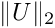, U might be real or complex

template<typename VEC1 , typename VEC2 >
scalar_type asm_L2_dist (const mesh_im &mim, const mesh_fem &mf1, const VEC1 &U1, const mesh_fem &mf2, const VEC2 &U2, mesh_region rg=mesh_region::all_convexes())
Compute the distance between U1 and U2, defined on two different mesh_fems (but sharing the same mesh), without interpolating U1 on mf2.

template<typename VEC >
scalar_type asm_H1_semi_norm (const mesh_im &mim, const mesh_fem &mf, const VEC &U, const mesh_region &rg=mesh_region::all_convexes())
compute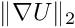, U might be real or complex

template<typename VEC1 , typename VEC2 >
scalar_type asm_H1_semi_dist (const mesh_im &mim, const mesh_fem &mf1, const VEC1 &U1, const mesh_fem &mf2, const VEC2 &U2, mesh_region rg=mesh_region::all_convexes())
Compute the H1 semi-distance between U1 and U2, defined on two different mesh_fems (but sharing the same mesh), without interpolating U1 on mf2.

template<typename VEC >
scalar_type asm_H1_norm (const mesh_im &mim, const mesh_fem &mf, const VEC &U, const mesh_region &rg=mesh_region::all_convexes())
compute the H1 norm of U. More...

template<typename VEC1 , typename VEC2 >
scalar_type asm_H1_dist (const mesh_im &mim, const mesh_fem &mf1, const VEC1 &U1, const mesh_fem &mf2, const VEC2 &U2, mesh_region rg=mesh_region::all_convexes())
Compute the H1 distance between U1 and U2.

template<typename VEC >
scalar_type asm_H2_semi_norm (const mesh_im &mim, const mesh_fem &mf, const VEC &U, const mesh_region &rg=mesh_region::all_convexes())
compute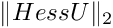, U might be real or complex. More...

template<typename VEC >
scalar_type asm_H2_norm (const mesh_im &mim, const mesh_fem &mf, const VEC &U, const mesh_region &rg=mesh_region::all_convexes())
compute the H2 norm of U (for C^1 elements).

template<typename VEC1 , typename VEC2 >
scalar_type asm_H2_dist (const mesh_im &mim, const mesh_fem &mf1, const VEC1 &U1, const mesh_fem &mf2, const VEC2 &U2, const mesh_region &rg=mesh_region::all_convexes())
Compute the H2 distance between U1 and U2.

template<typename MAT >
void asm_mass_matrix (const MAT &M, const mesh_im &mim, const mesh_fem &mf1, const mesh_region &rg=mesh_region::all_convexes())
generic mass matrix assembly (on the whole mesh or on the specified convex set or boundary)

template<typename MAT >
void asm_mass_matrix (const MAT &M, const mesh_im &mim, const mesh_fem &mf1, const mesh_fem &mf2, const mesh_region &rg=mesh_region::all_convexes())
generic mass matrix assembly (on the whole mesh or on the specified boundary)

template<typename MAT , typename VECT >
void asm_mass_matrix_param (const MAT &M, const mesh_im &mim, const mesh_fem &mf1, const mesh_fem &mf2, const mesh_fem &mf_data, const VECT &A, const mesh_region &rg=mesh_region::all_convexes())
generic mass matrix assembly with an additional parameter (on the whole mesh or on the specified boundary)

template<typename MAT , typename VECT >
void asm_mass_matrix_param (MAT &M, const mesh_im &mim, const mesh_fem &mf_u, const mesh_fem &mf_data, const VECT &F, const mesh_region &rg=mesh_region::all_convexes())
generic mass matrix assembly with an additional parameter (on the whole mesh or on the specified boundary)

template<typename MAT , typename VECT >
void asm_mass_matrix_homogeneous_param (MAT &M, const mesh_im &mim, const mesh_fem &mf_u, const VECT &F, const mesh_region &rg=mesh_region::all_convexes())
generic mass matrix assembly with an additional constant parameter (on the whole mesh or on the specified boundary)

template<typename MAT >
void asm_lumped_mass_matrix_for_first_order_from_consistent (const MAT &M)
lumped mass matrix assembly from consistent mass matrix

template<typename MAT >
void asm_lumped_mass_matrix_for_first_order (const MAT &M, const mesh_im &mim, const mesh_fem &mf1, const mesh_region &rg=mesh_region::all_convexes())
lumped mass matrix assembly (on the whole mesh or on the specified boundary)

template<typename MAT , typename VECT >
void asm_lumped_mass_matrix_for_first_order_param (MAT &M, const mesh_im &mim, const mesh_fem &mf_u, const mesh_fem &mf_data, const VECT &F, const mesh_region &rg=mesh_region::all_convexes())
lumped mass matrix assembly with an additional parameter (on the whole mesh or on the specified boundary)

template<typename VECT1 , typename VECT2 >
void asm_source_term (const VECT1 &B, const mesh_im &mim, const mesh_fem &mf, const mesh_fem &mf_data, const VECT2 &F, const mesh_region &rg=mesh_region::all_convexes())
source term (for both volumic sources and boundary (Neumann) sources).

template<typename VECT1 , typename VECT2 >
void asm_homogeneous_source_term (const VECT1 &B, const mesh_im &mim, const mesh_fem &mf, const VECT2 &F, const mesh_region &rg=mesh_region::all_convexes())
source term (for both volumic sources and boundary (Neumann) sources). More...

template<typename VECT1 , typename VECT2 >
void asm_normal_source_term (VECT1 &B, const mesh_im &mim, const mesh_fem &mf, const mesh_fem &mf_data, const VECT2 &F, const mesh_region &rg)
Normal source term (for boundary (Neumann) condition).

template<typename VECT1 , typename VECT2 >
void asm_homogeneous_normal_source_term (VECT1 &B, const mesh_im &mim, const mesh_fem &mf, const VECT2 &F, const mesh_region &rg)
Homogeneous normal source term (for boundary (Neumann) condition).

template<typename MAT , typename VECT >
void asm_qu_term (MAT &M, const mesh_im &mim, const mesh_fem &mf_u, const mesh_fem &mf_d, const VECT &Q, const mesh_region &rg)
assembly of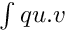More...

template<class MAT , class VECT >
void asm_stiffness_matrix_for_linear_elasticity (const MAT &M, const mesh_im &mim, const mesh_fem &mf, const mesh_fem &mf_data, const VECT &LAMBDA, const VECT &MU, const mesh_region &rg=mesh_region::all_convexes())
Stiffness matrix for linear elasticity, with Lamé coefficients.

template<class MAT , class VECT >
void asm_stiffness_matrix_for_homogeneous_linear_elasticity (const MAT &M, const mesh_im &mim, const mesh_fem &mf, const VECT &LAMBDA, const VECT &MU, const mesh_region &rg=mesh_region::all_convexes())
Stiffness matrix for linear elasticity, with constant Lamé coefficients.

template<typename MAT , typename VECT >
void asm_stiffness_matrix_for_linear_elasticity_Hooke (MAT &RM, const mesh_im &mim, const mesh_fem &mf, const mesh_fem &mf_data, const VECT &H, const mesh_region &rg=mesh_region::all_convexes())
Stiffness matrix for linear elasticity, with a general Hooke tensor. More...

template<typename MAT >
void asm_stokes_B (const MAT &B, const mesh_im &mim, const mesh_fem &mf_u, const mesh_fem &mf_p, const mesh_region &rg=mesh_region::all_convexes())
Build the mixed pressure term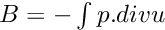.

template<typename MAT >
void asm_stiffness_matrix_for_homogeneous_laplacian (const MAT &M, const mesh_im &mim, const mesh_fem &mf, const mesh_region &rg=mesh_region::all_convexes())
assembly of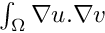.

template<typename MAT >
void asm_stiffness_matrix_for_homogeneous_laplacian_componentwise (const MAT &M, const mesh_im &mim, const mesh_fem &mf, const mesh_region &rg=mesh_region::all_convexes())
assembly of.

template<typename MAT , typename VECT >
void asm_stiffness_matrix_for_laplacian (MAT &M, const mesh_im &mim, const mesh_fem &mf, const mesh_fem &mf_data, const VECT &A, const mesh_region &rg=mesh_region::all_convexes())
assembly of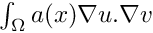, where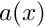is scalar.

template<typename MAT , typename VECT >
void asm_stiffness_matrix_for_laplacian_componentwise (MAT &M, const mesh_im &mim, const mesh_fem &mf, const mesh_fem &mf_data, const VECT &A, const mesh_region &rg=mesh_region::all_convexes())
The same as getfem::asm_stiffness_matrix_for_laplacian , but on each component of mf when mf has a qdim > 1.

template<typename MAT , typename VECT >
void asm_stiffness_matrix_for_scalar_elliptic (MAT &M, const mesh_im &mim, const mesh_fem &mf, const mesh_fem &mf_data, const VECT &A, const mesh_region &rg=mesh_region::all_convexes())
assembly of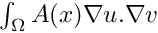, where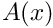is a (symmetric positive definite) NxN matrix. More...

template<typename MAT , typename VECT >
void asm_stiffness_matrix_for_homogeneous_scalar_elliptic (MAT &M, const mesh_im &mim, const mesh_fem &mf, const VECT &A, const mesh_region &rg=mesh_region::all_convexes())
The same but with a constant matrix.

template<typename MAT , typename VECT >
void asm_stiffness_matrix_for_scalar_elliptic_componentwise (MAT &M, const mesh_im &mim, const mesh_fem &mf, const mesh_fem &mf_data, const VECT &A, const mesh_region &rg=mesh_region::all_convexes())
The same but on each component of mf when mf has a qdim > 1.

template<typename MAT , typename VECT >
void asm_stiffness_matrix_for_homogeneous_scalar_elliptic_componentwise (MAT &M, const mesh_im &mim, const mesh_fem &mf, const VECT &A, const mesh_region &rg=mesh_region::all_convexes())
The same but with a constant matrix.

template<typename MAT , typename VECT >
void asm_stiffness_matrix_for_vector_elliptic (MAT &M, const mesh_im &mim, const mesh_fem &mf, const mesh_fem &mf_data, const VECT &A, const mesh_region &rg=mesh_region::all_convexes())
Assembly of, whereis a NxNxQxQ (symmetric positive definite) tensor defined on mf_data.

template<typename MAT , typename VECT >
void asm_stiffness_matrix_for_homogeneous_vector_elliptic (MAT &M, const mesh_im &mim, const mesh_fem &mf, const VECT &A, const mesh_region &rg=mesh_region::all_convexes())
Assembly of, whereis a NxNxQxQ (symmetric positive definite) constant tensor.

template<typename MAT , typename VECT >
void asm_Helmholtz (MAT &M, const mesh_im &mim, const mesh_fem &mf_u, const mesh_fem &mf_data, const VECT &K_squared, const mesh_region &rg=mesh_region::all_convexes())
assembly of the term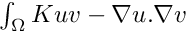, for the helmholtz equation (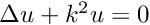, with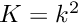). More...

template<typename MAT , typename VECT >
void asm_homogeneous_Helmholtz (MAT &M, const mesh_im &mim, const mesh_fem &mf_u, const VECT &K_squared, const mesh_region &rg=mesh_region::all_convexes())
assembly of the term, for the helmholtz equation (, with). More...

template<typename MAT , typename VECT1 , typename VECT2 >
void asm_dirichlet_constraints (MAT &H, VECT1 &R, const mesh_im &mim, const mesh_fem &mf_u, const mesh_fem &mf_mult, const mesh_fem &mf_r, const VECT2 &r_data, const mesh_region &region, int version=ASMDIR_BUILDALL)
Assembly of Dirichlet constraints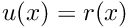in a weak form. More...

template<typename MAT , typename VECT1 , typename VECT2 , typename VECT3 >
void asm_generalized_dirichlet_constraints (MAT &H, VECT1 &R, const mesh_im &mim, const mesh_fem &mf_u, const mesh_fem &mf_h, const mesh_fem &mf_r, const VECT2 &h_data, const VECT3 &r_data, const mesh_region &region, int version=ASMDIR_BUILDALL)
Assembly of generalized Dirichlet constraints h(x)u(x) = r(x), where h is a QxQ matrix field (Q == mf_u.get_qdim()), outputs a (under-determined) linear system HU=R. More...

template<typename MAT1 , typename MAT2 , typename VECT1 , typename VECT2 >
size_type Dirichlet_nullspace (const MAT1 &H, MAT2 &NS, const VECT1 &R, VECT2 &U0)
Build an orthogonal basis of the kernel of H in NS, gives the solution of minimal norm of H*U = R in U0 and return the dimension of the kernel. More...

void add_raytracing_transformation (model &md, const std::string &transname, scalar_type release_distance)
Add a raytracing interpolate transformation called 'transname' to a model to be used by the generic assembly bricks.

void add_raytracing_transformation (ga_workspace &workspace, const std::string &transname, scalar_type release_distance)
Add a raytracing interpolate transformation called 'transname' to a workspace to be used by the generic assembly bricks.

void add_master_contact_boundary_to_raytracing_transformation (model &md, const std::string &transname, const mesh &m, const std::string &dispname, size_type region)
Add a master boundary with corresponding displacement variable 'dispname' on a specific boundary 'region' to an existing raytracing interpolate transformation called 'transname'.

void add_slave_contact_boundary_to_raytracing_transformation (model &md, const std::string &transname, const mesh &m, const std::string &dispname, size_type region)
Add a slave boundary with corresponding displacement variable 'dispname' on a specific boundary 'region' to an existing raytracing interpolate transformation called 'transname'.

void add_master_contact_boundary_to_raytracing_transformation (ga_workspace &workspace, const std::string &transname, const mesh &m, const std::string &dispname, size_type region)
Add a master boundary with corresponding displacement variable 'dispname' on a specific boundary 'region' to an existing raytracing interpolate transformation called 'transname'.

void add_slave_contact_boundary_to_raytracing_transformation (ga_workspace &workspace, const std::string &transname, const mesh &m, const std::string &dispname, size_type region)
Add a slave boundary with corresponding displacement variable 'dispname' on a specific boundary 'region' to an existing raytracing interpolate transformation called 'transname'.

void add_rigid_obstacle_to_raytracing_transformation (model &md, const std::string &transname, const std::string &expr, size_type N)
Add a rigid obstacle whose geometry corresponds to the zero level-set of the high-level generic assembly expression expr to an existing raytracing interpolate transformation called 'transname'. More...

void add_rigid_obstacle_to_raytracing_transformation (ga_workspace &workspace, const std::string &transname, const std::string &expr, size_type N)
Add a rigid obstacle whose geometry corresponds to the zero level-set of the high-level generic assembly expression 'expr' to an existing raytracing interpolate transformation called 'transname'.

void add_projection_transformation (model &md, const std::string &transname, scalar_type release_distance)
Add a projection interpolate transformation called 'transname' to a model to be used by the generic assembly bricks.

void add_projection_transformation (ga_workspace &workspace, const std::string &transname, scalar_type release_distance)
Add a projection interpolate transformation called 'transname' to a workspace to be used by the generic assembly bricks.

void add_master_contact_boundary_to_projection_transformation (model &md, const std::string &transname, const mesh &m, const std::string &dispname, size_type region)
Add a master boundary with corresponding displacement variable 'dispname' on a specific boundary 'region' to an existing projection interpolate transformation called 'transname'.

void add_master_contact_boundary_to_projection_transformation (ga_workspace &workspace, const std::string &transname, const mesh &m, const std::string &dispname, size_type region)
Add a master boundary with corresponding displacement variable 'dispname' on a specific boundary 'region' to an existing projection interpolate transformation called 'transname'.

void add_slave_contact_boundary_to_projection_transformation (model &md, const std::string &transname, const mesh &m, const std::string &dispname, size_type region)
Add a slave boundary with corresponding displacement variable 'dispname' on a specific boundary 'region' to an existing projection interpolate transformation called 'transname'.

void add_slave_contact_boundary_to_projection_transformation (ga_workspace &workspace, const std::string &transname, const mesh &m, const std::string &dispname, size_type region)
Add a slave boundary with corresponding displacement variable 'dispname' on a specific boundary 'region' to an existing projection interpolate transformation called 'transname'.

void add_rigid_obstacle_to_projection_transformation (model &md, const std::string &transname, const std::string &expr, size_type N)
Add a rigid obstacle whose geometry corresponds to the zero level-set of the high-level generic assembly expression expr to an existing projection interpolate transformation called 'transname'. More...

void add_rigid_obstacle_to_projection_transformation (ga_workspace &workspace, const std::string &transname, const std::string &expr, size_type N)
Add a rigid obstacle whose geometry corresponds to the zero level-set of the high-level generic assembly expression 'expr' to an existing projection interpolate transformation called 'transname'.

size_type add_integral_contact_with_rigid_obstacle_brick (model &md, const mesh_im &mim, const std::string &varname_u, const std::string &multname_n, const std::string &dataname_obs, const std::string &dataname_r, size_type region, int option=1)
Add a frictionless contact condition with a rigid obstacle to the model, which is defined in an integral way. More...

size_type add_integral_contact_with_rigid_obstacle_brick (model &md, const mesh_im &mim, const std::string &varname_u, const std::string &multname, const std::string &dataname_obs, const std::string &dataname_r, const std::string &dataname_friction_coeffs, size_type region, int option=1, const std::string &dataname_alpha="", const std::string &dataname_wt="", const std::string &dataname_gamma="", const std::string &dataname_vt="")
Add a contact with friction condition with a rigid obstacle to the model, which is defined in an integral way. More...

size_type add_penalized_contact_with_rigid_obstacle_brick (model &md, const mesh_im &mim, const std::string &varname_u, const std::string &dataname_obs, const std::string &dataname_r, size_type region, int option=1, const std::string &dataname_lambda_n="")
Add a penalized contact frictionless condition with a rigid obstacle to the model. More...

size_type add_penalized_contact_with_rigid_obstacle_brick (model &md, const mesh_im &mim, const std::string &varname_u, const std::string &dataname_obs, const std::string &dataname_r, const std::string &dataname_friction_coeffs, size_type region, int option=1, const std::string &dataname_lambda="", const std::string &dataname_alpha="", const std::string &dataname_wt="")
Add a penalized contact condition with Coulomb friction with a rigid obstacle to the model. More...

size_type add_integral_contact_between_nonmatching_meshes_brick (model &md, const mesh_im &mim, const std::string &varname_u1, const std::string &varname_u2, const std::string &multname_n, const std::string &dataname_r, size_type region1, size_type region2, int option=1)
Add a frictionless contact condition between nonmatching meshes to the model. More...

size_type add_integral_contact_between_nonmatching_meshes_brick (model &md, const mesh_im &mim, const std::string &varname_u1, const std::string &varname_u2, const std::string &multname, const std::string &dataname_r, const std::string &dataname_friction_coeffs, size_type region1, size_type region2, int option=1, const std::string &dataname_alpha="", const std::string &dataname_wt1="", const std::string &dataname_wt2="")
Add a contact with friction condition between nonmatching meshes to the model. More...

size_type add_penalized_contact_between_nonmatching_meshes_brick (model &md, const mesh_im &mim, const std::string &varname_u1, const std::string &varname_u2, const std::string &dataname_r, size_type region1, size_type region2, int option=1, const std::string &dataname_lambda_n="")
Add a penalized contact frictionless condition between nonmatching meshes to the model. More...

size_type add_penalized_contact_between_nonmatching_meshes_brick (model &md, const mesh_im &mim, const std::string &varname_u1, const std::string &varname_u2, const std::string &dataname_r, const std::string &dataname_friction_coeffs, size_type region1, size_type region2, int option=1, const std::string &dataname_lambda="", const std::string &dataname_alpha="", const std::string &dataname_wt1="", const std::string &dataname_wt2="")
Add a penalized contact condition with Coulomb friction between nonmatching meshes to the model. More...

template<typename VECT1 >
void asm_integral_contact_Uzawa_proj (VECT1 &R, const mesh_im &mim, const getfem::mesh_fem &mf_u, const VECT1 &U, const getfem::mesh_fem &mf_obs, const VECT1 &obs, const getfem::mesh_fem &mf_lambda, const VECT1 &lambda, const getfem::mesh_fem *pmf_coeff, const VECT1 &f_coeff, const VECT1 *WT, scalar_type r, scalar_type alpha, const mesh_region &rg, int option=1)
Specific assembly procedure for the use of an Uzawa algorithm to solve contact with rigid obstacle problems with friction.

template<typename VECT1 >
void asm_integral_contact_Uzawa_proj (VECT1 &R, const mesh_im &mim, const getfem::mesh_fem &mf_u, const VECT1 &U, const getfem::mesh_fem &mf_obs, const VECT1 &obs, const getfem::mesh_fem &mf_lambda, const VECT1 &lambda, scalar_type r, const mesh_region &rg)
Specific assembly procedure for the use of an Uzawa algorithm to solve contact problems.

template<typename VEC >
void asm_level_set_normal_source_term (VEC &R, const mesh_im &mim, const getfem::mesh_fem &mf_u, const getfem::mesh_fem &mf_obs, const VEC &obs, const getfem::mesh_fem &mf_lambda, const VEC &lambda, const mesh_region &rg)
Specific assembly procedure for the use of an Uzawa algorithm to solve contact problems.

size_type add_Nitsche_contact_with_rigid_obstacle_brick (model &md, const mesh_im &mim, const std::string &varname_u, const std::string &Neumannterm, const std::string &expr_obs, const std::string &dataname_gamma0, scalar_type theta_, std::string dataexpr_friction_coeff, const std::string &dataname_alpha, const std::string &dataname_wt, size_type region)
Adds a contact condition with or without Coulomb friction on the variable varname_u and the mesh boundary region. More...

size_type add_Nitsche_fictitious_domain_contact_brick (model &md, const mesh_im &mim, const std::string &varname_u1, const std::string &varname_u2, const std::string &dataname_d1, const std::string &dataname_d2, const std::string &dataname_gamma0, scalar_type theta, const std::string &dataname_friction_coeff, const std::string &dataname_alpha, const std::string &dataname_wt1, const std::string &dataname_wt2)
Adds a contact condition with or without Coulomb friction between two bodies in a fictitious domain. More...

size_type add_integral_large_sliding_contact_brick_raytracing (model &md, const std::string &augm_param, scalar_type release_distance, const std::string &f_coeff="0", const std::string &alpha="1", bool sym_v=false, bool frame_indifferent=false)
Adds a large sliding contact with friction brick to the model. More...

void add_contact_boundary_to_large_sliding_contact_brick (model &md, size_type indbrick, const mesh_im &mim, size_type region, bool is_master, bool is_slave, const std::string &u, const std::string &lambda="", const std::string &w="")
Adds a contact boundary to an existing large sliding contact with friction brick. More...

void add_rigid_obstacle_to_large_sliding_contact_brick (model &md, size_type indbrick, const std::string &expr, size_type N)
Adds a rigid obstacle to an existing large sliding contact with friction brick. More...

const std::string & sliding_data_group_name_of_large_sliding_contact_brick (model &md, size_type indbrick)
Gives the name of the group of variables corresponding to the sliding data for an existing large sliding contact brick.

const std::string & displacement_group_name_of_large_sliding_contact_brick (model &md, size_type indbrick)
Gives the name of the group of variables corresponding to the displacement for an existing large sliding contact brick.

const std::string & transformation_name_of_large_sliding_contact_brick (model &md, size_type indbrick)
Gives the name of the raytracing interpolate transformation for an existing large sliding contact brick.

size_type add_integral_large_sliding_contact_brick_raytrace (model &md, multi_contact_frame &mcf, const std::string &dataname_r, const std::string &dataname_friction_coeff=std::string(), const std::string &dataname_alpha=std::string())
Adds a large sliding contact with friction brick to the model. More...

size_type add_Nitsche_large_sliding_contact_brick_raytracing (model &md, bool is_unbiased, const std::string &Nitsche_param, scalar_type release_distance, const std::string &f_coeff="0", const std::string &alpha="1", bool sym_v=false, bool frame_indifferent=false)
Adds a large sliding contact with friction brick to the model based on Nitsche's method. More...

void add_contact_boundary_to_Nitsche_large_sliding_contact_brick (model &md, size_type indbrick, const mesh_im &mim, size_type region, bool is_master, bool is_slave, bool is_unbiased, const std::string &u, const std::string &lambda="", const std::string &w="")
Adds a contact boundary to an existing large sliding contact with friction brick. More...

void add_rigid_obstacle_to_Nitsche_large_sliding_contact_brick (model &md, size_type indbrick, const std::string &expr, size_type N)
Adds a rigid obstacle to an existing large sliding contact with friction brick. More...

const std::string & sliding_data_group_name_of_Nitsche_large_sliding_contact_brick (model &md, size_type indbrick)
Gives the name of the group of variables corresponding to the sliding data for an existing large sliding contact brick.

const std::string & displacement_group_name_of_Nitsche_large_sliding_contact_brick (model &md, size_type indbrick)
Gives the name of the group of variables corresponding to the displacement for an existing large sliding contact brick.

const std::string & transformation_name_of_Nitsche_large_sliding_contact_brick (model &md, size_type indbrick)
Gives the name of the raytracing interpolate transformation for an existing large sliding contact brick.

size_type add_basic_contact_brick (model &md, const std::string &varname_u, const std::string &multname_n, const std::string &dataname_r, CONTACT_B_MATRIX &BN, std::string dataname_gap="", std::string dataname_alpha="", int aug_version=1, bool Hughes_stabilized=false)
Add a frictionless contact condition to the model. More...

size_type add_basic_contact_brick_two_deformable_bodies (model &md, const std::string &varname_u1, const std::string &varname_u2, const std::string &multname_n, const std::string &dataname_r, CONTACT_B_MATRIX &BN1, CONTACT_B_MATRIX &BN2, std::string dataname_gap="", std::string dataname_alpha="", int aug_version=1, bool Hughes_stabilized=false)
Add a frictionless contact condition to the model between two deformable bodies. More...

size_type add_basic_contact_brick (model &md, const std::string &varname_u, const std::string &multname_n, const std::string &multname_t, const std::string &dataname_r, CONTACT_B_MATRIX &BN, CONTACT_B_MATRIX &BT, std::string dataname_friction_coeff, std::string dataname_gap="", std::string dataname_alpha="", int aug_version=1, bool Tresca_version=false, const std::string dataname_threshold="", std::string dataname_gamma="", std::string dataname_wt="", bool Hughes_stabilized=false)
Add a contact with friction condition to the model. More...

CONTACT_B_MATRIX & contact_brick_set_BN (model &md, size_type indbrick)
Can be used to change the matrix BN of a basic contact/friction brick.

void contact_brick_set_stationary (model &md, size_type indbrick)
Can be used to set the stationary option.

CONTACT_B_MATRIX & contact_brick_set_DN (model &md, size_type indbrick)
Can be used to change the matrix DN of a basic contact/friction brick.

CONTACT_B_MATRIX & contact_brick_set_DT (model &md, size_type indbrick)
Can be used to change the matrix DT of a basic contact/friction brick.

CONTACT_B_MATRIX & contact_brick_set_BT (model &md, size_type indbrick)
Can be used to change the matrix BT of a basic contact/friction brick.

size_type add_Hughes_stab_basic_contact_brick (model &md, const std::string &varname_u, const std::string &multname_n, const std::string &dataname_r, CONTACT_B_MATRIX &BN, CONTACT_B_MATRIX &DN, std::string dataname_gap="", std::string dataname_alpha="", int aug_version=1)
Add Hughes stabilized frictionless contact condition to the model. More...

size_type add_Hughes_stab_basic_contact_brick (model &md, const std::string &varname_u, const std::string &multname_n, const std::string &multname_t, const std::string &dataname_r, CONTACT_B_MATRIX &BN, CONTACT_B_MATRIX &BT, CONTACT_B_MATRIX &DN, CONTACT_B_MATRIX &DT, std::string dataname_friction_coeff, std::string dataname_gap="", std::string dataname_alpha="", int aug_version=1, bool Tresca_version=false, const std::string dataname_threshold="")
Add Hughes stabilized friction contact condition to the model (broken ?). More...

size_type add_nodal_contact_with_rigid_obstacle_brick (model &md, const mesh_im &mim, const std::string &varname_u, const std::string &multname_n, const std::string &dataname_r, size_type region, const std::string &obstacle, int aug_version=1)
Add a frictionless contact condition with a rigid obstacle to the model. More...

size_type add_nodal_contact_with_rigid_obstacle_brick (model &md, const mesh_im &mim, const std::string &varname_u, const std::string &multname_n, const std::string &multname_t, const std::string &dataname_r, const std::string &dataname_friction_coeff, size_type region, const std::string &obstacle, int aug_version=1)
Add a contact with friction condition with a rigid obstacle to the model. More...

size_type add_nodal_contact_between_nonmatching_meshes_brick (model &md, const mesh_im &mim1, const mesh_im &mim2, const std::string &varname_u1, const std::string &varname_u2, std::string &multname_n, const std::string &dataname_r, const std::vector< size_type > &rg1, const std::vector< size_type > &rg2, bool slave1=true, bool slave2=false, int aug_version=1)
Add a frictionless contact condition between two faces of one or two elastic bodies. More...

size_type add_nodal_contact_between_nonmatching_meshes_brick (model &md, const mesh_im &mim1, const mesh_im &mim2, const std::string &varname_u1, const std::string &varname_u2, std::string &multname_n, std::string &multname_t, const std::string &dataname_r, const std::string &dataname_friction_coeff, const std::vector< size_type > &rg1, const std::vector< size_type > &rg2, bool slave1=true, bool slave2=false, int aug_version=1)
Add a contact with friction condition between two faces of one or two elastic bodies. More...

template<class VECT1 , class VECT2 >
void convect (const mesh_fem &mf, VECT1 &U, const mesh_fem &mf_v, const VECT2 &V, scalar_type dt, size_type nt, convect_boundary_option option=CONVECT_EXTRAPOLATION, const base_node &per_min=base_node(), const base_node &per_max=base_node())
Compute the convection of a quantity on a getfem::mesh_fem with respect to a velocity field. More...

template<class VECT1 , class VECT2 >
void compute_gradient (const mesh_fem &mf, const mesh_fem &mf_target, const VECT1 &UU, VECT2 &VV)
Compute the gradient of a field on a getfem::mesh_fem. More...

template<class VECT1 , class VECT2 >
void compute_hessian (const mesh_fem &mf, const mesh_fem &mf_target, const VECT1 &UU, VECT2 &VV)
Compute the hessian of a field on a getfem::mesh_fem. More...

template<typename VEC1 , typename VEC2 >
void interpolation_von_mises (const getfem::mesh_fem &mf_u, const getfem::mesh_fem &mf_vm, const VEC1 &U, VEC2 &VM, scalar_type mu=1)
Compute the Von-Mises stress of a field (only valid for linearized elasticity in 3D)

template<typename VEC1 , typename VEC2 , typename VEC3 >
void interpolation_von_mises_or_tresca (const getfem::mesh_fem &mf_u, const getfem::mesh_fem &mf_vm, const VEC1 &U, VEC2 &VM, const getfem::mesh_fem &mf_lambda, const VEC3 &lambda, const getfem::mesh_fem &mf_mu, const VEC3 &mu, bool tresca)
Compute the Von-Mises stress of a field (valid for linearized elasticity in 2D and 3D)

pdof_description lagrange_dof (dim_type d)
Description of a unique dof of lagrange type (value at the node). More...

pdof_description derivative_dof (dim_type d, dim_type r)
Description of a unique dof of derivative type. More...

pdof_description second_derivative_dof (dim_type d, dim_type num_der1, dim_type num_der2)
Description of a unique dof of second derivative type. More...

pdof_description normal_derivative_dof (dim_type d)
Description of a unique dof of normal derivative type (normal derivative at the node, regarding a face). More...

pdof_description mean_value_dof (dim_type d)
Description of a unique dof of mean value type. More...

pdof_description global_dof (dim_type d)
Description of a global dof, i.e. More...

pdof_description product_dof (pdof_description pnd1, pdof_description pnd2)
Product description of the descriptions *pnd1 and *pnd2.

pdof_description xfem_dof (pdof_description p, size_type ind)
Description of a special dof for Xfem.

size_type dof_xfem_index (pdof_description)
Returns the xfem_index of dof (0 for normal dof)

int dof_description_compare (pdof_description a, pdof_description b)
Gives a total order on the dof description compatible with the identification.

Says if the dof is linkable.

bool dof_compatibility (pdof_description, pdof_description)
Says if the two dofs can be identified.

pfem classical_fem (bgeot::pgeometric_trans pgt, short_type k, bool complete=false)
Give a pointer on the structures describing the classical polynomial fem of degree k on a given convex type. More...

pfem classical_discontinuous_fem (bgeot::pgeometric_trans pg, short_type k, scalar_type alpha=0, bool complete=false)
Give a pointer on the structures describing the classical polynomial discontinuous fem of degree k on a given convex type. More...

pfem fem_descriptor (const std::string &name)
get a fem descriptor from its string name.

std::string name_of_fem (pfem p)
get the string name of a fem descriptor.

pfem_precomp fem_precomp (pfem pf, bgeot::pstored_point_tab pspt, dal::pstatic_stored_object dep)
Handles precomputations for FEM. More...

void delete_fem_precomp (pfem_precomp pfp)
Request for the removal of a pfem_precomp.

pfem interior_fem_of_hho_method (pfem hho_method)
Specific function for a HHO method to obtain the method in the interior. More...

pfem new_fem_global_function (const std::vector< pglobal_function > &funcs, const mesh &m)
create a new global function FEM. More...

pfem new_fem_global_function (const std::vector< pglobal_function > &funcs, const mesh_im &mim)
create a new global function FEM. More...

void del_fem_global_function (const pfem &pf)
release a global function FEM

template<typename MAT , typename VECT >
void asm_stiffness_matrix_for_bilaplacian (const MAT &M, const mesh_im &mim, const mesh_fem &mf, const mesh_fem &mf_data, const VECT &A, const mesh_region &rg=mesh_region::all_convexes())
assembly of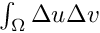.

size_type add_bilaplacian_brick (model &md, const mesh_im &mim, const std::string &varname, const std::string &dataname, size_type region=size_type(-1))
Adds a bilaplacian brick on the variable varname and on the mesh region region. More...

size_type add_bilaplacian_brick_KL (model &md, const mesh_im &mim, const std::string &varname, const std::string &dataname1, const std::string &dataname2, size_type region=size_type(-1))
Adds a bilaplacian brick on the variable varname and on the mesh region region. More...

template<typename VECT1 , typename VECT2 >
void asm_normal_derivative_source_term (VECT1 &B, const mesh_im &mim, const mesh_fem &mf, const mesh_fem &mf_data, const VECT2 &F, const mesh_region &rg)
assembly of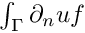.

size_type add_normal_derivative_source_term_brick (model &md, const mesh_im &mim, const std::string &varname, const std::string &dataname, size_type region)
Adds a normal derivative source term brick :math:F = \int b.  More...

size_type add_Kirchhoff_Love_Neumann_term_brick (model &md, const mesh_im &mim, const std::string &varname, const std::string &dataname1, const std::string &dataname2, size_type region)
Adds a Neumann term brick for Kirchhoff-Love model on the variable varname and the mesh region region. More...

template<typename MAT , typename VECT1 , typename VECT2 >
void asm_normal_derivative_dirichlet_constraints (MAT &H, VECT1 &R, const mesh_im &mim, const mesh_fem &mf_u, const mesh_fem &mf_mult, const mesh_fem &mf_r, const VECT2 &r_data, const mesh_region &rg, bool R_must_be_derivated, int version)
Assembly of normal derivative Dirichlet constraints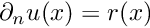in a weak form. More...

size_type add_normal_derivative_Dirichlet_condition_with_multipliers (model &md, const mesh_im &mim, const std::string &varname, const std::string &multname, size_type region, const std::string &dataname=std::string(), bool R_must_be_derivated=false)
Adds a Dirichlet condition on the normal derivative of the variable varname and on the mesh region region (which should be a boundary). More...

size_type add_normal_derivative_Dirichlet_condition_with_multipliers (model &md, const mesh_im &mim, const std::string &varname, const mesh_fem &mf_mult, size_type region, const std::string &dataname=std::string(), bool R_must_be_derivated=false)
Adds a Dirichlet condition on the normal derivative of the variable varname and on the mesh region region (which should be a boundary). More...

size_type add_normal_derivative_Dirichlet_condition_with_multipliers (model &md, const mesh_im &mim, const std::string &varname, dim_type degree, size_type region, const std::string &dataname=std::string(), bool R_must_be_derivated=false)
Adds a Dirichlet condition on the normal derivative of the variable varname and on the mesh region region (which should be a boundary). More...

size_type add_normal_derivative_Dirichlet_condition_with_penalization (model &md, const mesh_im &mim, const std::string &varname, scalar_type penalisation_coeff, size_type region, const std::string &dataname=std::string(), bool R_must_be_derivated=false)
Adds a Dirichlet condition on the normal derivative of the variable varname and on the mesh region region (which should be a boundary). More...

void ga_local_projection (const getfem::model &md, const mesh_im &mim, const std::string &expr, const mesh_fem &mf, base_vector &result, const mesh_region &rg=mesh_region::all_convexes())
Make an elementwise L2 projection of an expression with respect to the mesh_fem mf. More...

void add_interpolate_transformation_from_expression (ga_workspace &workspace, const std::string &transname, const mesh &source_mesh, const mesh &target_mesh, const std::string &expr)
Add a transformation to a workspace workspace or a model md mapping point in mesh source_mesh to mesh target_mesh, optionally restricted to the region target_region. More...

void add_interpolate_transformation_on_deformed_domains (ga_workspace &workspace, const std::string &transname, const mesh &source_mesh, const std::string &source_displacements, const mesh_region &source_region, const mesh &target_mesh, const std::string &target_displacements, const mesh_region &target_region)
Add a transformation to the workspace that creates an identity mapping between two meshes in deformed state. More...

void add_interpolate_transformation_on_deformed_domains (model &md, const std::string &transname, const mesh &source_mesh, const std::string &source_displacements, const mesh_region &source_region, const mesh &target_mesh, const std::string &target_displacements, const mesh_region &target_region)
The same as above, but adding transformation to the model. More...

pinterpolate_transformation interpolate_transformation_neighbor_instance ()
Create a new instance of a transformation corresponding to the interpolation on the neighbor element. More...

Add to the model the elementary transformation corresponding to the reconstruction of a gradient for HHO methods. More...

Add to the model the elementary transformation corresponding to the reconstruction of a symmetrized gradient for HHO methods. More...

void add_HHO_reconstructed_value (model &md, std::string name)
Add to the model the elementary transformation corresponding to the reconstruction of the variable. More...

void add_HHO_reconstructed_symmetrized_value (model &md, std::string name)
Add to the model the elementary transformation corresponding to the reconstruction of the variable using a symmetrized gradient. More...

void add_HHO_stabilization (model &md, std::string name)
Add to the model the elementary transformation corresponding to the HHO stabilization operator. More...

void add_HHO_symmetrized_stabilization (model &md, std::string name)
Add to the model the elementary transformation corresponding to the HHO stabilization operator using a symmetrized gradient. More...

bool is_equivalent_with_vector (const bgeot::multi_index &sizes, size_type vector_size)
check if a given tensor size is equivalent to a vector

bool is_equivalent_with_matrix (const bgeot::multi_index &sizes, size_type nrows, size_type ncols)
check if a given tensor size is equivalent to a matrix

void import_mesh (const std::string &filename, const std::string &format, mesh &m)
imports a mesh file. More...

void import_mesh_gmsh (const std::string &filename, mesh &m, std::map< std::string, size_type > &region_map, bool remove_last_dimension=true, std::map< size_type, std::set< size_type >> *nodal_map=NULL, bool remove_duplicated_nodes=true)
Import a mesh file in format generated by Gmsh. More...

void maybe_remove_last_dimension (mesh &msh)
for gmsh and gid meshes, the mesh nodes are always 3D, so for a 2D mesh the z-component of nodes should be removed

std::map< std::string, size_type > read_region_names_from_gmsh_mesh_file (std::istream &f)
for gmsh meshes, create table linking region name to region_id.

pintegration_method int_method_descriptor (std::string name, bool throw_if_not_found=true)
Get an integration method from its name . More...

std::string name_of_int_method (pintegration_method p)
Get the string name of an integration method .

pintegration_method classical_exact_im (bgeot::pgeometric_trans pgt)
return an exact integration method for convex type handled by pgt. More...

pintegration_method classical_approx_im (bgeot::pgeometric_trans pgt, dim_type degree)
try to find an approximate integration method for the geometric transformation pgt which is able to integrate exactly polynomials of degree <= "degree". More...

pintegration_method exact_simplex_im (size_type n)
return IM_EXACT_SIMPLEX(n)

pintegration_method exact_parallelepiped_im (size_type n)
return IM_EXACT_PARALLELEPIPED(n)

pintegration_method exact_prism_im (size_type n)
return IM_EXACT_PRISM(n)

pintegration_method exact_classical_im (bgeot::pgeometric_trans pgt) IS_DEPRECATED

pintegration_method im_none (void)
return IM_NONE

pfem new_interpolated_fem (const mesh_fem &mef, const mesh_im &mim, pinterpolated_func pif=0, dal::bit_vector blocked_dof=dal::bit_vector(), bool store_val=true)
create a new interpolated FEM. More...

void del_interpolated_fem (const pfem &pf)
release an interpolated fem

template<typename VECT , typename F >
void interpolation_function (mesh_fem &mf_target, const VECT &VV, F &f, mesh_region rg=mesh_region::all_convexes())
interpolation of a function f on mf_target. More...

template<typename VECTU , typename VECTV >
void interpolation (const mesh_fem &mf_source, const mesh_fem &mf_target, const VECTU &U, VECTV &V, int extrapolation=0, double EPS=1E-10, mesh_region rg_source=mesh_region::all_convexes(), mesh_region rg_target=mesh_region::all_convexes())
interpolation/extrapolation of (mf_source, U) on mf_target. More...

template<typename MAT >
void interpolation (const mesh_fem &mf_source, const mesh_fem &mf_target, MAT &M, int extrapolation=0, double EPS=1E-10, mesh_region rg_source=mesh_region::all_convexes(), mesh_region rg_target=mesh_region::all_convexes())
Build the interpolation matrix of mf_source on mf_target. More...

template<typename VECT >
void interpolation_to_im_data (const mesh_fem &mf_source, const im_data &im_target, const VECT &nodal_data, VECT &int_pt_data, bool use_im_data_filter=true)
Interpolate mesh_fem data to im_data. More...

void add_2D_rotated_RT0_projection (model &md, std::string name)
Add the elementary transformation corresponding to the projection on rotated RT0 element for two-dimensional elements to the model. More...

void add_P0_projection (model &md, std::string name)
Add the elementary transformation corresponding to the projection on P0 element. More...

size_type add_Mindlin_Reissner_plate_brick (model &md, const mesh_im &mim, const mesh_im &mim_reduced, const std::string &u3, const std::string &Theta, const std::string &param_E, const std::string &param_nu, const std::string &param_epsilon, const std::string &param_kappa, size_type variant=size_type(2), size_type region=size_type(-1))
Add a term corresponding to the classical Reissner-Mindlin plate model for which u3 is the transverse displacement, Theta the rotation of fibers normal to the midplane, 'param_E' the Young Modulus, param_nu the poisson ratio, param_epsilon the plate thickness, param_kappa the shear correction factor. More...

size_type add_enriched_Mindlin_Reissner_plate_brick (model &md, const mesh_im &mim, const mesh_im &mim_reduced1, const mesh_im &mim_reduced2, const std::string &ua, const std::string &Theta, const std::string &u3, const std::string &Theta3, const std::string &param_E, const std::string &param_nu, const std::string &param_epsilon, size_type variant=size_type(3), size_type region=size_type(-1))
Add a term corresponding to the enriched Reissner-Mindlin plate model for which varname_ua is the membrane displacements, varname_u3 is the transverse displacement, varname_theta the rotation of fibers normal to the midplane, varname_theta3 the pinching,
'param_E' the Young Modulus, param_nu the poisson ratio, param_epsilon the plate thickness. More...

pmat_elem_computation mat_elem (pmat_elem_type pm, pintegration_method pi, bgeot::pgeometric_trans pg, bool prefer_comp_on_real_element=false)
allocate a structure for computation (integration over elements or faces of elements) of elementary tensors. More...

void APIDECL extrude (const mesh &in, mesh &out, size_type nb_layers, short_type degree=short_type(1))
build a N+1 dimensions mesh from a N-dimensions mesh by extrusion.

scalar_type APIDECL convex_area_estimate (bgeot::pgeometric_trans pgt, const base_matrix &pts, pintegration_method pim)
rough estimate of the convex area. More...

scalar_type APIDECL convex_quality_estimate (bgeot::pgeometric_trans pgt, const base_matrix &pts)
rough estimate of the maximum value of the condition number of the jacobian of the geometric transformation

scalar_type APIDECL convex_radius_estimate (bgeot::pgeometric_trans pgt, const base_matrix &pts)
rough estimate of the radius of the convex using the largest eigenvalue of the jacobian of the geometric transformation

void APIDECL outer_faces_of_mesh (const mesh &m, const dal::bit_vector &cvlst, convex_face_ct &flist)
returns a list of "exterior" faces of a mesh (i.e. More...

mesh_region APIDECL inner_faces_of_mesh (const mesh &m, const mesh_region &mr=mesh_region::all_convexes())
Select all the faces sharing at least two element of the given mesh region. More...

mesh_region APIDECL all_faces_of_mesh (const mesh &m, const mesh_region &mr=mesh_region::all_convexes())
Select all the faces of the given mesh region. More...

mesh_region APIDECL select_faces_of_normal (const mesh &m, const mesh_region &mr, const base_small_vector &V, scalar_type angle)
Select in the region mr the faces of the mesh m with their unit outward vector having a maximal angle "angle" with the vector V.

mesh_region APIDECL select_faces_in_box (const mesh &m, const mesh_region &mr, const base_node &pt1, const base_node &pt2)
Select in the region mr the faces of the mesh m lying entirely in the box delimated by pt1 and pt2.

mesh_region APIDECL select_faces_in_ball (const mesh &m, const mesh_region &mr, const base_node &center, scalar_type radius)
Select in the region mr the faces of the mesh m lying entirely in the ball delimated by pt1 and radius.

const mesh_femclassical_mesh_fem (const mesh &mesh, dim_type degree, dim_type qdim=1, bool complete=false)
Gives the descriptor of a classical finite element method of degree K on mesh. More...

const mesh_femdummy_mesh_fem ()
Dummy mesh_fem for default parameter of functions.

template<typename VEC1 , typename VEC2 >
void slice_vector_on_basic_dof_of_element (const mesh_fem &mf, const VEC1 &vec, size_type cv, VEC2 &coeff, size_type qmult1=size_type(-1), size_type qmult2=size_type(-1))
Given a mesh_fem. More...

const mesh_imdummy_mesh_im ()
Dummy mesh_im for default parameter of functions.

const mesh_regiondummy_mesh_region ()
Dummy mesh_region for default parameter of functions.

void standard_solve (model &md, gmm::iteration &iter, rmodel_plsolver_type lsolver, abstract_newton_line_search &ls)
A default solver for the model brick system. More...

bool is_old (const std::string &name)
Does the variable have Old_ prefix.

std::string no_old_prefix_name (const std::string &name)
Strip the variable name from prefix Old_ if it has one.

void APIDECL add_theta_method_dispatcher (model &md, dal::bit_vector ibricks, const std::string &THETA)
Add a theta-method time dispatcher to a list of bricks. More...

void APIDECL velocity_update_for_order_two_theta_method (model &md, const std::string &U, const std::string &V, const std::string &pdt, const std::string &ptheta)
Function which udpate the velocity $v^{n+1}$ after the computation of the displacement $u^{n+1}$ and before the next iteration. More...

void APIDECL add_midpoint_dispatcher (model &md, dal::bit_vector ibricks)
Add a midpoint time dispatcher to a list of bricks. More...

void APIDECL velocity_update_for_Newmark_scheme (model &md, size_type id2dt2b, const std::string &U, const std::string &V, const std::string &pdt, const std::string &ptwobeta, const std::string &pgamma)
Function which udpate the velocity $v^{n+1}$ after the computation of the displacement $u^{n+1}$ and before the next iteration. More...

size_type APIDECL add_linear_term (model &md, const mesh_im &mim, const std::string &expr, size_type region=size_type(-1), bool is_sym=false, bool is_coercive=false, const std::string &brickname="", bool return_if_nonlin=false)
Add a term given by the weak form language expression expr which will be assembled in region region and with the integration method mim. More...

size_type APIDECL add_nonlinear_term (model &md, const mesh_im &mim, const std::string &expr, size_type region=size_type(-1), bool is_sym=false, bool is_coercive=false, const std::string &brickname="")
Add a nonlinear term given by the weak form language expression expr which will be assembled in region region and with the integration method mim. More...

size_type APIDECL add_source_term (model &md, const mesh_im &mim, const std::string &expr, size_type region=size_type(-1), const std::string &brickname=std::string(), const std::string &directvarname=std::string(), const std::string &directdataname=std::string(), bool return_if_nonlin=false)
Add a source term given by the assembly string expr which will be assembled in region region and with the integration method mim. More...

size_type APIDECL add_linear_twodomain_term (model &md, const mesh_im &mim, const std::string &expr, size_type region, const std::string &secondary_domain, bool is_sym=false, bool is_coercive=false, const std::string &brickname="", bool return_if_nonlin=false)
Adds a linear term given by a weak form language expression like add_linear_term function but for an integration on a direct product of two domains, a first specfied by mim and region and a second one by secondary_domain which has to be declared first into the model.

size_type APIDECL add_nonlinear_twodomain_term (model &md, const mesh_im &mim, const std::string &expr, size_type region, const std::string &secondary_domain, bool is_sym=false, bool is_coercive=false, const std::string &brickname="")
Adds a nonlinear term given by a weak form language expression like add_nonlinear_term function but for an integration on a direct product of two domains, a first specfied by mim and region and a second one by secondary_domain which has to be declared first into the model.

size_type APIDECL add_twodomain_source_term (model &md, const mesh_im &mim, const std::string &expr, size_type region, const std::string &secondary_domain, const std::string &brickname=std::string(), const std::string &directvarname=std::string(), const std::string &directdataname=std::string(), bool return_if_nonlin=false)
Adds a source term given by a weak form language expression like add_source_term function but for an integration on a direct product of two domains, a first specfied by mim and region and a second one by secondary_domain which has to be declared first into the model.

size_type APIDECL add_Laplacian_brick (model &md, const mesh_im &mim, const std::string &varname, size_type region=size_type(-1))
Add a Laplacian term on the variable varname (in fact with a minus : :math:-\text{div}(\nabla u)). More...

size_type APIDECL add_generic_elliptic_brick (model &md, const mesh_im &mim, const std::string &varname, const std::string &dataexpr, size_type region=size_type(-1))
Add an elliptic term on the variable varname. More...

size_type APIDECL add_source_term_brick (model &md, const mesh_im &mim, const std::string &varname, const std::string &dataexpr, size_type region=size_type(-1), const std::string &directdataname=std::string())
Add a source term on the variable varname. More...

size_type APIDECL add_normal_source_term_brick (model &md, const mesh_im &mim, const std::string &varname, const std::string &dataexpr, size_type region)
Add a source term on the variable varname on a boundary region. More...

size_type APIDECL add_Dirichlet_condition_with_simplification (model &md, const std::string &varname, size_type region, const std::string &dataname=std::string())
Add a (simple) Dirichlet condition on the variable varname and the mesh region region. More...

size_type APIDECL add_Dirichlet_condition_with_multipliers (model &md, const mesh_im &mim, const std::string &varname, const std::string &multname, size_type region, const std::string &dataname=std::string())
Add a Dirichlet condition on the variable varname and the mesh region region. More...

size_type APIDECL add_Dirichlet_condition_with_multipliers (model &md, const mesh_im &mim, const std::string &varname, const mesh_fem &mf_mult, size_type region, const std::string &dataname=std::string())
Same function as the previous one but the multipliers variable will be declared to the brick by the function. More...

size_type APIDECL add_Dirichlet_condition_with_multipliers (model &md, const mesh_im &mim, const std::string &varname, dim_type degree, size_type region, const std::string &dataname=std::string())
Same function as the previous one but the mf_mult parameter is replaced by degree. More...

const APIDECL std::string & mult_varname_Dirichlet (model &md, size_type ind_brick)
When ind_brick is the index of a Dirichlet brick with multiplier on the model md, the function return the name of the multiplier variable. More...

size_type APIDECL add_Dirichlet_condition_with_penalization (model &md, const mesh_im &mim, const std::string &varname, scalar_type penalization_coeff, size_type region, const std::string &dataname=std::string(), const mesh_fem *mf_mult=0)
Add a Dirichlet condition on the variable varname and the mesh region region. More...

size_type APIDECL add_Dirichlet_condition_with_Nitsche_method (model &md, const mesh_im &mim, const std::string &varname, const std::string &Neumannterm, const std::string &datagamma0, size_type region, scalar_type theta=scalar_type(0), const std::string &datag=std::string())
Add a Dirichlet condition on the variable varname and the mesh region region. More...

size_type APIDECL add_normal_Dirichlet_condition_with_multipliers (model &md, const mesh_im &mim, const std::string &varname, const std::string &multname, size_type region, const std::string &dataname=std::string())
Add a Dirichlet condition to the normal component of the vector (or tensor) valued variable varname and the mesh region region. More...

size_type APIDECL add_normal_Dirichlet_condition_with_multipliers (model &md, const mesh_im &mim, const std::string &varname, const mesh_fem &mf_mult, size_type region, const std::string &dataname=std::string())
Same function as the previous one but the multipliers variable will be declared to the brick by the function. More...

size_type APIDECL add_normal_Dirichlet_condition_with_multipliers (model &md, const mesh_im &mim, const std::string &varname, dim_type degree, size_type region, const std::string &dataname=std::string())
Same function as the previous one but the mf_mult parameter is replaced by degree. More...

size_type APIDECL add_normal_Dirichlet_condition_with_penalization (model &md, const mesh_im &mim, const std::string &varname, scalar_type penalization_coeff, size_type region, const std::string &dataname=std::string(), const mesh_fem *mf_mult=0)
Add a Dirichlet condition to the normal component of the vector (or tensor) valued variable varname and the mesh region region. More...

size_type APIDECL add_normal_Dirichlet_condition_with_Nitsche_method (model &md, const mesh_im &mim, const std::string &varname, const std::string &Neumannterm, const std::string &datagamma0, size_type region, scalar_type theta=scalar_type(0), const std::string &datag=std::string())
Add a Dirichlet condition on the normal component of the variable varname and the mesh region region. More...

size_type APIDECL add_pointwise_constraints_with_penalization (model &md, const std::string &varname, scalar_type penalisation_coeff, const std::string &dataname_pt, const std::string &dataname_unitv=std::string(), const std::string &dataname_val=std::string())
Add some pointwise constraints on the variable varname thanks to a penalization. More...

size_type APIDECL add_pointwise_constraints_with_given_multipliers (model &md, const std::string &varname, const std::string &multname, const std::string &dataname_pt, const std::string &dataname_unitv=std::string(), const std::string &dataname_val=std::string())
Add some pointwise constraints on the variable varname using a given multiplier multname. More...

size_type APIDECL add_pointwise_constraints_with_multipliers (model &md, const std::string &varname, const std::string &dataname_pt, const std::string &dataname_unitv=std::string(), const std::string &dataname_val=std::string())
Add some pointwise constraints on the variable varname using multiplier. More...

void APIDECL change_penalization_coeff (model &md, size_type ind_brick, scalar_type penalisation_coeff)
Change the penalization coefficient of a Dirichlet condition with penalization brick. More...

size_type APIDECL add_generalized_Dirichlet_condition_with_multipliers (model &md, const mesh_im &mim, const std::string &varname, const std::string &multname, size_type region, const std::string &dataname, const std::string &Hname)
Add a generalized Dirichlet condition on the variable varname and the mesh region region. More...

size_type APIDECL add_generalized_Dirichlet_condition_with_multipliers (model &md, const mesh_im &mim, const std::string &varname, const mesh_fem &mf_mult, size_type region, const std::string &dataname, const std::string &Hname)
Same function as the preceeding one but the multipliers variable will be declared to the brick by the function. More...

size_type APIDECL add_generalized_Dirichlet_condition_with_multipliers (model &md, const mesh_im &mim, const std::string &varname, dim_type degree, size_type region, const std::string &dataname, const std::string &Hname)
Same function as the preceeding one but the mf_mult parameter is replaced by degree. More...

size_type APIDECL add_generalized_Dirichlet_condition_with_penalization (model &md, const mesh_im &mim, const std::string &varname, scalar_type penalization_coeff, size_type region, const std::string &dataname, const std::string &Hname, const mesh_fem *mf_mult=0)
Add a Dirichlet condition on the variable varname and the mesh region region. More...

size_type APIDECL add_generalized_Dirichlet_condition_with_Nitsche_method (model &md, const mesh_im &mim, const std::string &varname, const std::string &Neumannterm, const std::string &datagamma0, size_type region, scalar_type theta, const std::string &datag, const std::string &dataH)
Add a Dirichlet condition on the variable varname and the mesh region region. More...

size_type APIDECL add_Helmholtz_brick (model &md, const mesh_im &mim, const std::string &varname, const std::string &dataexpr, size_type region=size_type(-1))
Add a Helmoltz brick to the model. More...

size_type APIDECL add_Fourier_Robin_brick (model &md, const mesh_im &mim, const std::string &varname, const std::string &dataexpr, size_type region)
Add a Fourier-Robin brick to the model. More...

template<typename VECT >
void set_private_data_rhs (model &md, size_type indbrick, const VECT &L)
For some specific bricks having an internal right hand side vector (explicit bricks: 'constraint brick' and 'explicit rhs brick'), set this rhs.

template<typename MAT >
void set_private_data_matrix (model &md, size_type indbrick, const MAT &B)
For some specific bricks having an internal sparse matrix (explicit bricks: 'constraint brick' and 'explicit matrix brick'), set this matrix. More...

template<typename MAT , typename VECT >
size_type add_constraint_with_penalization (model &md, const std::string &varname, scalar_type penalisation_coeff, const MAT &B, const VECT &L)
Add an additional explicit penalized constraint on the variable varname. More...

template<typename MAT , typename VECT >
size_type add_constraint_with_multipliers (model &md, const std::string &varname, const std::string &multname, const MAT &B, const VECT &L)
Add an additional explicit constraint on the variable varname thank to a multiplier multname peviously added to the model (should be a fixed size variable). More...

template<typename MAT >
size_type add_explicit_matrix (model &md, const std::string &varname1, const std::string &varname2, const MAT &B, bool issymmetric=false, bool iscoercive=false)
Add a brick reprenting an explicit matrix to be added to the tangent linear system relatively to the variables 'varname1' and 'varname2'. More...

template<typename VECT >
size_type add_explicit_rhs (model &md, const std::string &varname, const VECT &L)
Add a brick representing an explicit right hand side to be added to the right hand side of the tangent linear system relatively to the variable 'varname'. More...

size_type APIDECL add_isotropic_linearized_elasticity_brick (model &md, const mesh_im &mim, const std::string &varname, const std::string &dataname_lambda, const std::string &dataname_mu, size_type region=size_type(-1), const std::string &dataname_preconstraint=std::string())
Linear elasticity brick (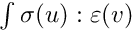). More...

size_type APIDECL add_isotropic_linearized_elasticity_pstrain_brick (model &md, const mesh_im &mim, const std::string &varname, const std::string &data_E, const std::string &data_nu, size_type region)
Linear elasticity brick (). More...

size_type APIDECL add_isotropic_linearized_elasticity_pstress_brick (model &md, const mesh_im &mim, const std::string &varname, const std::string &data_E, const std::string &data_nu, size_type region)
Linear elasticity brick (). More...

template<class VECTVM >
void compute_isotropic_linearized_Von_Mises_or_Tresca (model &md, const std::string &varname, const std::string &dataname_lambda, const std::string &dataname_mu, const mesh_fem &mf_vm, VECTVM &VM, bool tresca)
Compute the Von-Mises stress or the Tresca stress of a field (only valid for isotropic linearized elasticity in 3D) Parametrized by Lame coefficients.

void APIDECL compute_isotropic_linearized_Von_Mises_pstrain (model &md, const std::string &varname, const std::string &data_E, const std::string &data_nu, const mesh_fem &mf_vm, model_real_plain_vector &VM)
Compute the Von-Mises stress of a displacement field for isotropic linearized elasticity in 3D or in 2D with plane strain assumption. More...

void APIDECL compute_isotropic_linearized_Von_Mises_pstress (model &md, const std::string &varname, const std::string &data_E, const std::string &data_nu, const mesh_fem &mf_vm, model_real_plain_vector &VM)
Compute the Von-Mises stress of a displacement field for isotropic linearized elasticity in 3D or in 2D with plane stress assumption. More...

size_type APIDECL add_linear_incompressibility (model &md, const mesh_im &mim, const std::string &varname, const std::string &multname_pressure, size_type region=size_type(-1), const std::string &dataexpr_penal_coeff=std::string())
Mixed linear incompressibility condition brick. More...

size_type APIDECL add_mass_brick (model &md, const mesh_im &mim, const std::string &varname, const std::string &dataexpr_rho=std::string(), size_type region=size_type(-1))
Mass brick (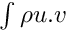). More...

size_type APIDECL add_lumped_mass_for_first_order_brick (model &md, const mesh_im &mim, const std::string &varname, const std::string &dataexpr_rho=std::string(), size_type region=size_type(-1))
Lumped mass brick for first order. More...

size_type APIDECL add_basic_d_on_dt_brick (model &md, const mesh_im &mim, const std::string &varname, const std::string &dataname_dt, const std::string &dataname_rho=std::string(), size_type region=size_type(-1))
Basic d/dt brick (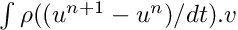). More...

size_type APIDECL add_basic_d2_on_dt2_brick (model &md, const mesh_im &mim, const std::string &varnameU, const std::string &datanameV, const std::string &dataname_dt, const std::string &dataname_alpha, const std::string &dataname_rho=std::string(), size_type region=size_type(-1))
Basic d2/dt2 brick (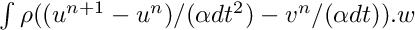). More...

template<typename MAT , typename VECT >
void asm_navier_stokes_tgm (const MAT &M, const mesh_im &mim, const mesh_fem &mf, const VECT &U, const mesh_region &rg=mesh_region::all_convexes())
assembly of Tangent matrix for Navier-Stokes.

template<typename VECT1 , typename VECT2 >
void asm_navier_stokes_rhs (const VECT1 &V, const mesh_im &mim, const mesh_fem &mf, const VECT2 &U, const mesh_region &rg=mesh_region::all_convexes())
assembly of right hand side for Navier-Stokes.

template<typename MAT , typename VECT1 , typename VECT2 >
void asm_nonlinear_elasticity_tangent_matrix (const MAT &K_, const mesh_im &mim, const getfem::mesh_fem &mf, const VECT1 &U, const getfem::mesh_fem *mf_data, const VECT2 &PARAMS, const abstract_hyperelastic_law &AHL, const mesh_region &rg=mesh_region::all_convexes())
Tangent matrix for the non-linear elasticity.

size_type add_nonlinear_elasticity_brick (model &md, const mesh_im &mim, const std::string &varname, const phyperelastic_law &AHL, const std::string &dataname, size_type region=size_type(-1))
Add a nonlinear (large strain) elasticity term to the model with respect to the variable varname (deprecated brick, use add_finite_strain_elaticity instead). More...

template<class VECTVM >
void compute_Von_Mises_or_Tresca (model &md, const std::string &varname, const phyperelastic_law &AHL, const std::string &dataname, const mesh_fem &mf_vm, VECTVM &VM, bool tresca)
Compute the Von-Mises stress or the Tresca stress of a field with respect to the constitutive elasticity law AHL (only valid in 3D).

size_type add_nonlinear_incompressibility_brick (model &md, const mesh_im &mim, const std::string &varname, const std::string &multname, size_type region=size_type(-1))
Add a nonlinear incompressibility term (for large strain elasticity) to the model with respect to the variable varname (the displacement) and multname (the pressure).

size_type add_finite_strain_elasticity_brick (model &md, const mesh_im &mim, const std::string &lawname, const std::string &varname, const std::string &params, size_type region=size_type(-1))
Add a finite strain elasticity brick to the model with respect to the variable varname (the displacement). More...

size_type add_finite_strain_incompressibility_brick (model &md, const mesh_im &mim, const std::string &varname, const std::string &multname, size_type region=size_type(-1))
Add a finite strain incompressibility term (for large strain elasticity) to the model with respect to the variable varname (the displacement) and multname (the pressure). More...

void compute_finite_strain_elasticity_Von_Mises (model &md, const std::string &lawname, const std::string &varname, const std::string &params, const mesh_fem &mf_vm, model_real_plain_vector &VM, const mesh_region &rg=mesh_region::all_convexes())
Interpolate the Von-Mises stress of a field varname with respect to the nonlinear elasticity constitutive law lawname with parameters params (only valid in 3D).

set maximum number of OpenMP threads

is the program running in the parallel section

is the program is running on a single thread

size_type max_concurrency ()
Maximum number of threads that can run concurrently.

dal::bit_vector select_dofs_from_im (const mesh_fem &mf, const mesh_im &mim, unsigned P=unsigned(-1))
Return a selection of dof who contribute significantly to the mass-matrix that would be computed with mf and the integration method mim. More...

size_type add_small_strain_elastoplasticity_brick (model &md, const mesh_im &mim, std::string lawname, plasticity_unknowns_type unknowns_type, const std::vector< std::string > &varnames, const std::vector< std::string > &params, size_type region=size_type(-1))
Adds a small strain plasticity term to the model md. More...

void small_strain_elastoplasticity_next_iter (model &md, const mesh_im &mim, std::string lawname, plasticity_unknowns_type unknowns_type, const std::vector< std::string > &varnames, const std::vector< std::string > &params, size_type region=size_type(-1))
Function that allows to pass from a time step to another for the small strain plastic brick. More...

void compute_small_strain_elastoplasticity_Von_Mises (model &md, const mesh_im &mim, std::string lawname, plasticity_unknowns_type unknowns_type, const std::vector< std::string > &varnames, const std::vector< std::string > &params, const mesh_fem &mf_vm, model_real_plain_vector &VM, size_type region=size_type(-1))
This function computes the Von Mises stress field with respect to a small strain elastoplasticity term, approximated on mf_vm, and stores the result into VM. More...

void ga_define_linear_hardening_function (const std::string &name, scalar_type sigma_y0, scalar_type H, bool frobenius=true)
Add a linear function with the name specified by name to represent linear isotropoc hardening in plasticity with initial yield limit sigma_y0 and hardening modulus H. More...

void ga_define_Ramberg_Osgood_hardening_function (const std::string &name, scalar_type sigma_ref, scalar_type eps_ref, scalar_type n, bool frobenius=false)
Add a Ramberg-Osgood hardening function with the name specified by name, for reference stress and strain given by sigma_ref and eps_ref respectively and for a hardening exponent n. More...

void ga_define_Ramberg_Osgood_hardening_function (const std::string &name, scalar_type sigma_ref, scalar_type E, scalar_type alpha, scalar_type n, bool frobenius=false)
Add a Ramberg-Osgood hardening function with the name specified by name, for reference stress sigma_ref, Young's modulus E, offset parameter alpha and hardening parameter n. More...

size_type add_finite_strain_elastoplasticity_brick (model &md, const mesh_im &mim, std::string lawname, plasticity_unknowns_type unknowns_type, const std::vector< std::string > &varnames, const std::vector< std::string > &params, size_type region=size_type(-1))
Add a finite strain elastoplasticity brick to the model. More...

void finite_strain_elastoplasticity_next_iter (model &md, const mesh_im &mim, std::string lawname, plasticity_unknowns_type unknowns_type, const std::vector< std::string > &varnames, const std::vector< std::string > &params, size_type region=size_type(-1))
This function permits to update the state variables for a finite strain elastoplasticity brick, based on the current displacements and plastic multiplier fields (optionally also the the pressure field in the case of a mixed displacement-pressure formulation). More...

void compute_finite_strain_elastoplasticity_Von_Mises (model &md, const mesh_im &mim, std::string lawname, plasticity_unknowns_type unknowns_type, const std::vector< std::string > &varnames, const std::vector< std::string > &params, const mesh_fem &mf_vm, model_real_plain_vector &VM, size_type region=size_type(-1))
This function computes the Von Mises stress field with respect to a finite strain elastoplasticity term, approximated on mf_vm, and stores the result into VM. More...

size_type add_elastoplasticity_brick (model &md, const mesh_im &mim, const pconstraints_projection &ACP, const std::string &varname, const std::string &previous_dep_name, const std::string &datalambda, const std::string &datamu, const std::string &datathreshold, const std::string &datasigma, size_type region=size_type(-1))
Add a nonlinear elastoplasticity term to the model for small deformations and an isotropic material, with respect to the variable varname. More...

void elastoplasticity_next_iter (model &md, const mesh_im &mim, const std::string &varname, const std::string &previous_dep_name, const pconstraints_projection &ACP, const std::string &datalambda, const std::string &datamu, const std::string &datathreshold, const std::string &datasigma)
This function permits to compute the new stress constraints values supported by the material after a load or an unload. More...

void compute_elastoplasticity_Von_Mises_or_Tresca (model &md, const std::string &datasigma, const mesh_fem &mf_vm, model_real_plain_vector &VM, bool tresca)
This function computes on mf_vm the Von Mises or Tresca stress of a field for elastoplasticity and return it into the vector VM. More...

void compute_plastic_part (model &md, const mesh_im &mim, const mesh_fem &mf_pl, const std::string &varname, const std::string &previous_dep_name, const pconstraints_projection &ACP, const std::string &datalambda, const std::string &datamu, const std::string &datathreshold, const std::string &datasigma, model_real_plain_vector &plast)
This function computes on mf_pl the plastic part, that could appear after a load and an unload, into the vector plast. More...

pfem new_projected_fem (const mesh_fem &mf_source, const mesh_im &mim_target, size_type rg_source_=size_type(-1), size_type rg_target_=size_type(-1), dal::bit_vector blocked_dofs=dal::bit_vector(), bool store_val=true)
create a new projected FEM. More...

void del_projected_fem (pfem pf)
release a projected fem

void regular_unit_mesh (mesh &m, std::vector< size_type > nsubdiv, bgeot::pgeometric_trans pgt, bool noised=false)
Build a regular mesh of the unit square/cube/, etc. More...

void regular_mesh (mesh &m, const std::string &st)
Build a regular mesh parametrized by the string st. More...

void regular_ball_mesh (mesh &m, const std::string &st)
Build a regular mesh on a ball, parametrized by the string st. More...

void regular_ball_shell_mesh (mesh &m, const std::string &st)
Build a regular mesh on a ball shell, parametrized by the string st. More...

## Variables

const typedef fem< bgeot::base_poly > * ppolyfem
Classical polynomial FEM.

const typedef fem< bgeot::polynomial_composite > * ppolycompfem
Polynomial composite FEM.

const typedef fem< bgeot::base_rational_fraction > * prationalfracfem
Rational fration FEM.

const auto PREFIX_OLD = std::string{"Old_"}
A prefix to refer to the previous version of a variable.

## Detailed Description

GEneric Tool for Finite Element Methods.

Providing for special Math functions unavailable on Intel or MSVS C++ compilers.

## ◆ equal_resize()

template<class T >
 void getfem::equal_resize ( T & a, const T & b )

Resize 'a' to the same size as 'b'.

a and b can be matrices, vectors, or lists of matrices or vectors

Definition at line 147 of file getfem_accumulated_distro.h.

 void getfem::add_rigid_obstacle_to_raytracing_transformation ( model & md, const std::string & transname, const std::string & expr, size_type N )

Add a rigid obstacle whose geometry corresponds to the zero level-set of the high-level generic assembly expression expr to an existing raytracing interpolate transformation called 'transname'.

In expr, the current position is denoted X with components X(1), X(2), .... It is also allowed to use x instead of X(1), y instead of X(2), z instead of X(3) and w instead of X(4).

Definition at line 2480 of file getfem_contact_and_friction_common.cc.

 void getfem::add_rigid_obstacle_to_projection_transformation ( model & md, const std::string & transname, const std::string & expr, size_type N )

Add a rigid obstacle whose geometry corresponds to the zero level-set of the high-level generic assembly expression expr to an existing projection interpolate transformation called 'transname'.

In expr, the current position is denoted X with components X(1), X(2), .... It is also allowed to use x instead of X(1), y instead of X(2), z instead of X(3) and w instead of X(4).

Definition at line 2554 of file getfem_contact_and_friction_common.cc.

 size_type getfem::add_integral_contact_with_rigid_obstacle_brick ( model & md, const mesh_im & mim, const std::string & varname_u, const std::string & multname_n, const std::string & dataname_obs, const std::string & dataname_r, size_type region, int option = 1 )

Add a frictionless contact condition with a rigid obstacle to the model, which is defined in an integral way.

It is the direct approximation of an augmented Lagrangian formulation (see Getfem user documentation) defined at the continuous level. The advantage should be a better scalability: the number of Newton iterations should be more or less independent of the mesh size. The condition is applied on the variable varname_u on the boundary corresponding to region. The rigid obstacle should be described with the data dataname_obstacle being a signed distance to the obstacle (interpolated on a finite element method). multname_n should be a fem variable representing the contact stress. An inf-sup condition between multname_n and varname_u is required. The augmentation parameter dataname_r should be chosen in a range of acceptable values. Possible values for option is 1 for the non-symmetric Alart-Curnier augmented Lagrangian method, 2 for the symmetric one, 3 for the non-symmetric Alart-Curnier method with an additional augmentation. The default value is 1.

Definition at line 922 of file getfem_contact_and_friction_integral.cc.

 size_type getfem::add_integral_contact_with_rigid_obstacle_brick ( model & md, const mesh_im & mim, const std::string & varname_u, const std::string & multname, const std::string & dataname_obs, const std::string & dataname_r, const std::string & dataname_friction_coeffs, size_type region, int option = 1, const std::string & dataname_alpha = "", const std::string & dataname_wt = "", const std::string & dataname_gamma = "", const std::string & dataname_vt = "" )

Add a contact with friction condition with a rigid obstacle to the model, which is defined in an integral way.

It is the direct approximation of an augmented Lagrangian formulation (see Getfem user documentation) defined at the continuous level. The advantage should be a better scalability: the number of Newton iterations should be more or less independent of the mesh size. The condition is applied on the variable varname_u on the boundary corresponding to region. The rigid obstacle should be described with the data dataname_obstacle being a signed distance to the obstacle (interpolated on a finite element method). multname should be a fem variable representing the contact and friction stress. An inf-sup condition between multname and varname_u is required. The augmentation parameter dataname_r should be chosen in a range of acceptable values. The parameter dataname_friction_coeffs contains the Coulomb friction coefficient and optionally an adhesional shear stress threshold and the tresca limit shear stress. For constant coefficients its size is from 1 to 3. For coefficients described on a finite element method, this vector contains a number of single values, value pairs or triplets equal to the number of the corresponding mesh_fem's basic dofs. Possible values for option is 1 for the non-symmetric Alart-Curnier augmented Lagrangian method, 2 for the symmetric one, 3 for the non-symmetric Alart-Curnier method with an additional augmentation and 4 for a new unsymmetric method. The default value is 1. (Option 4 ignores any adhesional stress and tresca limit coefficients.) dataname_alpha and dataname_wt are optional parameters to solve evolutionary friction problems. dataname_gamma and dataname_vt represent optional data for adding a parameter-dependent sliding velocity to the friction condition.

Definition at line 962 of file getfem_contact_and_friction_integral.cc.

 size_type getfem::add_penalized_contact_with_rigid_obstacle_brick ( model & md, const mesh_im & mim, const std::string & varname_u, const std::string & dataname_obs, const std::string & dataname_r, size_type region, int option = 1, const std::string & dataname_lambda_n = "" )

Add a penalized contact frictionless condition with a rigid obstacle to the model.

The condition is applied on the variable varname_u on the boundary corresponding to region. The rigid obstacle should be described with the data dataname_obstacle being a signed distance to the obstacle (interpolated on a finite element method). The penalization parameter dataname_r should be chosen large enough to prescribe an approximate non-penetration condition but not too large not to deteriorate too much the conditionning of the tangent system. dataname_n is an optional parameter used if option is 2. In that case, the penalization term is shifted by lambda_n (this allows the use of an Uzawa algorithm on the corresponding augmented Lagrangian formulation)

Definition at line 1297 of file getfem_contact_and_friction_integral.cc.

 size_type getfem::add_penalized_contact_with_rigid_obstacle_brick ( model & md, const mesh_im & mim, const std::string & varname_u, const std::string & dataname_obs, const std::string & dataname_r, const std::string & dataname_friction_coeffs, size_type region, int option = 1, const std::string & dataname_lambda = "", const std::string & dataname_alpha = "", const std::string & dataname_wt = "" )

Add a penalized contact condition with Coulomb friction with a rigid obstacle to the model.

The condition is applied on the variable varname_u on the boundary corresponding to region. The rigid obstacle should be described with the data dataname_obstacle being a signed distance to the obstacle (interpolated on a finite element method). The parameter dataname_friction_coeffs contains the Coulomb friction coefficient and optionally an adhesional shear stress threshold and the tresca limit shear stress. For constant coefficients its size is from 1 to 3. For coefficients described on a finite element method, this vector contains a number of single values, value pairs or triplets equal to the number of the corresponding mesh_fem's basic dofs. The penalization parameter dataname_r should be chosen large enough to prescribe approximate non-penetration and friction conditions but not too large not to deteriorate too much the conditionning of the tangent system. dataname_lambda is an optional parameter used if option is 2. In that case, the penalization term is shifted by lambda (this allows the use of an Uzawa algorithm on the corresponding augmented Lagrangian formulation) dataname_alpha and dataname_wt are optional parameters to solve evolutionary friction problems.

Definition at line 1326 of file getfem_contact_and_friction_integral.cc.

 size_type getfem::add_integral_contact_between_nonmatching_meshes_brick ( model & md, const mesh_im & mim, const std::string & varname_u1, const std::string & varname_u2, const std::string & multname_n, const std::string & dataname_r, size_type region1, size_type region2, int option = 1 )

Add a frictionless contact condition between nonmatching meshes to the model.

This brick adds a contact which is defined in an integral way. It is the direct approximation of an augmented Lagrangian formulation (see Getfem user documentation) defined at the continuous level. The advantage should be a better scalability: the number of Newton iterations should be more or less independent of the mesh size. The condition is applied on the variables varname_u1 and varname_u2 on the boundaries corresponding to region1 and region2. multname_n should be a fem variable representing the contact stress. An inf-sup condition between multname_n and varname_u1 and varname_u2 is required. The augmentation parameter dataname_r should be chosen in a range of acceptable values. Possible values for option is 1 for the non-symmetric Alart-Curnier augmented Lagrangian method, 2 for the symmetric one, 3 for the non-symmetric Alart-Curnier method with an additional augmentation. The default value is 1.

Definition at line 1860 of file getfem_contact_and_friction_integral.cc.

 size_type getfem::add_integral_contact_between_nonmatching_meshes_brick ( model & md, const mesh_im & mim, const std::string & varname_u1, const std::string & varname_u2, const std::string & multname, const std::string & dataname_r, const std::string & dataname_friction_coeffs, size_type region1, size_type region2, int option = 1, const std::string & dataname_alpha = "", const std::string & dataname_wt1 = "", const std::string & dataname_wt2 = "" )

Add a contact with friction condition between nonmatching meshes to the model.

This brick adds a contact which is defined in an integral way. It is the direct approximation of an augmented Lagrangian formulation (see Getfem user documentation) defined at the continuous level. The advantage should be a better scalability: the number of Newton iterations should be more or less independent of the mesh size. The condition is applied on the variables varname_u1 and varname_u2 on the boundaries corresponding to region1 and region2. multname should be a fem variable representing the contact and friction stress. An inf-sup condition between multname and varname_u1 and varname_u2 is required. The augmentation parameter dataname_r should be chosen in a range of acceptable values. The parameter dataname_friction_coeffs contains the Coulomb friction coefficient and optionally an adhesional shear stress threshold and the tresca limit shear stress. For constant coefficients its size is from 1 to 3. For coefficients described on a finite element method on the same mesh as varname_u1, this vector contains a number of single values, value pairs or triplets equal to the number of the corresponding mesh_fem's basic dofs. Possible values for option is 1 for the non-symmetric Alart-Curnier augmented Lagrangian method, 2 for the symmetric one, 3 for the non-symmetric Alart-Curnier method with an additional augmentation and 4 for a new unsymmetric method. The default value is 1. (Option 4 ignores any adhesional stress and tresca limit coefficients.) dataname_alpha, dataname_wt1 and dataname_wt2 are optional parameters to solve evolutionary friction problems.

Definition at line 1906 of file getfem_contact_and_friction_integral.cc.

 size_type getfem::add_penalized_contact_between_nonmatching_meshes_brick ( model & md, const mesh_im & mim, const std::string & varname_u1, const std::string & varname_u2, const std::string & dataname_r, size_type region1, size_type region2, int option = 1, const std::string & dataname_lambda_n = "" )

Add a penalized contact frictionless condition between nonmatching meshes to the model.

The condition is applied on the variables varname_u1 and varname_u2 on the boundaries corresponding to region1 and region2. The penalization parameter dataname_r should be chosen large enough to prescribe an approximate non-penetration condition but not too large not to deteriorate too much the conditionning of the tangent system. dataname_n is an optional parameter used if option is 2. In that case, the penalization term is shifted by lambda_n (this allows the use of an Uzawa algorithm on the corresponding augmented Lagrangian formulation)

Definition at line 2360 of file getfem_contact_and_friction_integral.cc.

 size_type getfem::add_penalized_contact_between_nonmatching_meshes_brick ( model & md, const mesh_im & mim, const std::string & varname_u1, const std::string & varname_u2, const std::string & dataname_r, const std::string & dataname_friction_coeffs, size_type region1, size_type region2, int option = 1, const std::string & dataname_lambda = "", const std::string & dataname_alpha = "", const std::string & dataname_wt1 = "", const std::string & dataname_wt2 = "" )

Add a penalized contact condition with Coulomb friction between nonmatching meshes to the model.

The condition is applied on the variables varname_u1 and varname_u2 on the boundaries corresponding to region1 and region2. The penalization parameter dataname_r should be chosen large enough to prescribe approximate non-penetration and friction conditions but not too large not to deteriorate too much the conditionning of the tangent system. The parameter dataname_friction_coeffs contains the Coulomb friction coefficient and optionally an adhesional shear stress threshold and the tresca limit shear stress. For constant coefficients its size is from 1 to 3. For coefficients described on a finite element method on the same mesh as varname_u1, this vector contains a number of single values, value pairs or triplets equal to the number of the corresponding mesh_fem's basic dofs. dataname_lambda is an optional parameter used if option is 2. In that case, the penalization term is shifted by lambda (this allows the use of an Uzawa algorithm on the corresponding augmented Lagrangian formulation) dataname_alpha, dataname_wt1 and dataname_wt2 are optional parameters to solve evolutionary friction problems.

Definition at line 2392 of file getfem_contact_and_friction_integral.cc.

 size_type getfem::add_Nitsche_contact_with_rigid_obstacle_brick ( model & md, const mesh_im & mim, const std::string & varname_u, const std::string & Neumannterm, const std::string & expr_obs, const std::string & dataname_gamma0, scalar_type theta_, std::string dataexpr_friction_coeff, const std::string & dataname_alpha, const std::string & dataname_wt, size_type region )

Adds a contact condition with or without Coulomb friction on the variable varname_u and the mesh boundary region.

Neumannterm is the expression of the Neumann term (obtained by the Green formula) described as an expression of the high-level generic assembly language. This term can be obtained with md.Neumann_term(varname, region) once all volumic bricks have been added to the model. The contact condition is prescribed with Nitsche's method. The rigid obstacle should be described with the data dataname_obstacle being a signed distance to the obstacle (interpolated on a finite element method). gamma0name is the Nitsche's method parameter. theta is a scalar value which can be positive or negative. theta = 1 corresponds to the standard symmetric method which is conditionnaly coercive for gamma0 small. theta = -1 corresponds to the skew-symmetric method which is inconditionnaly coercive. theta = 0 is the simplest method for which the second derivative of the Neumann term is not necessary. The optional parameter dataexpr_friction_coeff is the friction coefficient which could be any expression of the assembly language. Returns the brick index in the model.

Definition at line 2544 of file getfem_contact_and_friction_integral.cc.

 size_type getfem::add_Nitsche_fictitious_domain_contact_brick ( model & md, const mesh_im & mim, const std::string & varname_u1, const std::string & varname_u2, const std::string & dataname_d1, const std::string & dataname_d2, const std::string & dataname_gamma0, scalar_type theta, const std::string & dataname_friction_coeff, const std::string & dataname_alpha, const std::string & dataname_wt1, const std::string & dataname_wt2 )

Adds a contact condition with or without Coulomb friction between two bodies in a fictitious domain.

The contact condition is applied on the variable varname_u1 corresponds with the first and slave body with Nitsche's method and on the variable varname_u2 corresponds with the second and master body with Nitsche's method. The contact condition is evaluated on the fictitious slave bondary. The first body should be described by the level-set dataname_d1 and the second body should be described by the level-set dataname_d2. gamma0name is the Nitsche's method parameter. theta is a scalar value which can be positive or negative. theta = 1 corresponds to the standard symmetric method which is conditionnaly coercive for gamma0 small. theta = -1 corresponds to the skew-symmetric method which is inconditionnaly coercive. theta = 0 is the simplest method for which the second derivative of the Neumann term is not necessary. The optional parameter dataname_friction_coeff is the friction coefficient which could be constant or defined on a finite element method. CAUTION: This brick has to be added in the model after all the bricks corresponding to partial differential terms having a Neumann term. Moreover, This brick can only be applied to bricks declaring their Neumann terms. Returns the brick index in the model.

 size_type getfem::add_integral_large_sliding_contact_brick_raytracing ( model & md, const std::string & augm_param, scalar_type release_distance, const std::string & f_coeff = "0", const std::string & alpha = "1", bool sym_v = false, bool frame_indifferent = false )

Adds a large sliding contact with friction brick to the model.

This brick is able to deal with self-contact, contact between several deformable bodies and contact with rigid obstacles. It uses the high-level generic assembly. It adds to the model a raytracing_interpolate_transformation object. For each slave boundary a multiplier variable should be defined. The release distance should be determined with care (generally a few times a mean element size, and less than the thickness of the body). Initially, the brick is added with no contact boundaries. The contact boundaries and rigid bodies are added with special functions.

Definition at line 2591 of file getfem_contact_and_friction_large_sliding.cc.

 void getfem::add_contact_boundary_to_large_sliding_contact_brick ( model & md, size_type indbrick, const mesh_im & mim, size_type region, bool is_master, bool is_slave, const std::string & u, const std::string & lambda = "", const std::string & w = "" )

Adds a contact boundary to an existing large sliding contact with friction brick.

When a boundary is declared slave, a multiplier lambda has to be given which whould be defined on the boundary region. The integration of contact terms is performed on each slave boundary. A boundary can be both declare master and slave which allows self-contact detection.

Definition at line 2529 of file getfem_contact_and_friction_large_sliding.cc.

 void getfem::add_rigid_obstacle_to_large_sliding_contact_brick ( model & md, size_type indbrick, const std::string & expr, size_type N )

Adds a rigid obstacle to an existing large sliding contact with friction brick.

expr is an expression using the high-level generic assembly language (where x is the current position) which should be a signed distance to the obstacle. N is the mesh dimension.

Definition at line 2518 of file getfem_contact_and_friction_large_sliding.cc.

 size_type getfem::add_integral_large_sliding_contact_brick_raytrace ( model & md, multi_contact_frame & mcf, const std::string & dataname_r, const std::string & dataname_friction_coeff = std::string(), const std::string & dataname_alpha = std::string() )

Adds a large sliding contact with friction brick to the model.

This brick is able to deal with self-contact, contact between several deformable bodies and contact with rigid obstacles. It takes a variable of type multi_contact_frame wich describe the contact situation (master and slave contact boundaries, self-contact detection or not, and a few parameter). For each slave boundary (and also master boundaries if self-contact is asked) a multiplier variable should be defined. DEPRECATED brick.

Definition at line 1137 of file getfem_contact_and_friction_large_sliding.cc.

 size_type getfem::add_Nitsche_large_sliding_contact_brick_raytracing ( model & md, bool is_unbiased, const std::string & Nitsche_param, scalar_type release_distance, const std::string & f_coeff = "0", const std::string & alpha = "1", bool sym_v = false, bool frame_indifferent = false )

Adds a large sliding contact with friction brick to the model based on Nitsche's method.

This brick is able to deal with self-contact, contact between several deformable bodies and contact with rigid obstacles. It uses the high-level generic assembly. It adds to the model a raytracing_interpolate_transformation object. For each slave boundary a material law should be defined as a function of the dispacement variable on this boundary. The release distance should be determined with care (generally a few times a mean element size, and less than the thickness of the body). Initially, the brick is added with no contact boundaries. The contact boundaries and rigid bodies are added with special functions.

Definition at line 2928 of file getfem_contact_and_friction_large_sliding.cc.

 void getfem::add_contact_boundary_to_Nitsche_large_sliding_contact_brick ( model & md, size_type indbrick, const mesh_im & mim, size_type region, bool is_master, bool is_slave, bool is_unbiased, const std::string & u, const std::string & lambda = "", const std::string & w = "" )

Adds a contact boundary to an existing large sliding contact with friction brick.

When a boundary is declared slave, a multiplier lambda has to be given which whould be defined on the boundary region. The integration of contact terms is performed on each slave boundary. A boundary can be both declare master and slave which allows self-contact detection.

Definition at line 2866 of file getfem_contact_and_friction_large_sliding.cc.

 void getfem::add_rigid_obstacle_to_Nitsche_large_sliding_contact_brick ( model & md, size_type indbrick, const std::string & expr, size_type N )

Adds a rigid obstacle to an existing large sliding contact with friction brick.

expr is an expression using the high-level generic assembly language (where x is the current position) which should be a signed distance to the obstacle. N is the mesh dimension.

Definition at line 2855 of file getfem_contact_and_friction_large_sliding.cc.

 size_type getfem::add_basic_contact_brick ( model & md, const std::string & varname_u, const std::string & multname_n, const std::string & dataname_r, CONTACT_B_MATRIX & BN, std::string dataname_gap = "", std::string dataname_alpha = "", int aug_version = 1, bool Hughes_stabilized = false )

Add a frictionless contact condition to the model.

If U is the vector of degrees of freedom on which the unilateral constraint is applied, the matrix BN has to be such that this condition is defined by $B_N U \le gap$. The constraint is prescribed thank to a multiplier multname_n whose dimension should be equal to the number of lines of BN. The augmentation parameter r should be chosen in a range of acceptabe values (see Getfem user documentation). dataname_gap is an optional parameter representing the initial gap. It can be a single value or a vector of value. dataname_alpha is an optional homogenization parameter for the augmentation parameter (see Getfem user documentation). The parameter aug_version indicates the augmentation strategy : 1 for the non-symmetric Alart-Curnier augmented Lagrangian, 2 for the symmetric one, 3 for the unsymmetric method with augmented multiplier.

Definition at line 1299 of file getfem_contact_and_friction_nodal.cc.

 size_type getfem::add_basic_contact_brick_two_deformable_bodies ( model & md, const std::string & varname_u1, const std::string & varname_u2, const std::string & multname_n, const std::string & dataname_r, CONTACT_B_MATRIX & BN1, CONTACT_B_MATRIX & BN2, std::string dataname_gap = "", std::string dataname_alpha = "", int aug_version = 1, bool Hughes_stabilized = false )

Add a frictionless contact condition to the model between two deformable bodies.

If U1, U2 are the vector of degrees of freedom on which the unilateral constraint is applied, the matrices BN1 and BN2 have to be such that this condition is defined by $B_{N1} U_1 B_{N2} U_2 + \le gap$. The constraint is prescribed thank to a multiplier multname_n whose dimension should be equal to the number of lines of BN. The augmentation parameter r should be chosen in a range of acceptabe values (see Getfem user documentation). dataname_gap is an optional parameter representing the initial gap. It can be a single value or a vector of value. dataname_alpha is an optional homogenization parameter for the augmentation parameter (see Getfem user documentation). The parameter aug_version indicates the augmentation strategy : 1 for the non-symmetric Alart-Curnier augmented Lagrangian, 2 for the symmetric one, 3 for the unsymmetric method with augmented multiplier.

Definition at line 1342 of file getfem_contact_and_friction_nodal.cc.

 size_type getfem::add_basic_contact_brick ( model & md, const std::string & varname_u, const std::string & multname_n, const std::string & multname_t, const std::string & dataname_r, CONTACT_B_MATRIX & BN, CONTACT_B_MATRIX & BT, std::string dataname_friction_coeff, std::string dataname_gap = "", std::string dataname_alpha = "", int aug_version = 1, bool Tresca_version = false, const std::string dataname_threshold = "", std::string dataname_gamma = "", std::string dataname_wt = "", bool Hughes_stabilized = false )

Add a contact with friction condition to the model.

If U is the vector of degrees of freedom on which the condition is applied, the matrix BN has to be such that the contact condition is defined by $B_N U \le gap$ and BT have to be such that the relative tangential displacement is $B_T U$. The matrix BT should have as many rows as BN multiplied by $d-1$ where $d$ is the domain dimension. The contact condition is prescribed thank to a multiplier multname_n whose dimension should be equal to the number of rows of BN and the friction condition by a mutliplier multname_t whose size should be the number of rows of BT. The parameter dataname_friction_coeff describes the friction coefficient. It could be a scalar or a vector describing the coefficient on each contact condition. The augmentation parameter r should be chosen in a range of acceptabe values (see Getfem user documentation). dataname_gap is an optional parameter representing the initial gap. It can be a single value or a vector of value. dataname_alpha is an optional homogenization parameter for the augmentation parameter (see Getfem user documentation). The parameter aug_version indicates the augmentation strategy : 1 for the non-symmetric Alart-Curnier augmented Lagrangian, 2 for the symmetric one, 3 for the unsymmetric method with augmented multiplier and 4 for the unsymmetric method with augmented multiplier and De Saxce projection.

Definition at line 1391 of file getfem_contact_and_friction_nodal.cc.

 size_type getfem::add_Hughes_stab_basic_contact_brick ( model & md, const std::string & varname_u, const std::string & multname_n, const std::string & dataname_r, CONTACT_B_MATRIX & BN, CONTACT_B_MATRIX & DN, std::string dataname_gap = "", std::string dataname_alpha = "", int aug_version = 1 )
inline

Add Hughes stabilized frictionless contact condition to the model.

If U is the vector of degrees of freedom on which the unilateral constraint is applied, and Lambda the multiplier Vector of contact force.Then Hughes stabilized frictionless contact condition is defined by the matrix BN and 'DN' have to be such that this condition is defined by $B_N U - DN Lambda \le 0$. where 'DN' is the masse matrix relative to stabilzed term. The augmentation parameter r should be chosen in a range of acceptabe values. dataname_gap is an optional parameter representing the initial gap. It can be a single value or a vector of value. dataname_alpha is an optional homogenization parameter for the augmentation parameter. The parameter aug_version indicates the augmentation strategy : 1 for the non-symmetric Alart-Curnier augmented Lagrangian, 2 for the symmetric one, 3 for the unsymmetric method with augmented multiplier.

Definition at line 167 of file getfem_contact_and_friction_nodal.h.

 size_type getfem::add_Hughes_stab_basic_contact_brick ( model & md, const std::string & varname_u, const std::string & multname_n, const std::string & multname_t, const std::string & dataname_r, CONTACT_B_MATRIX & BN, CONTACT_B_MATRIX & BT, CONTACT_B_MATRIX & DN, CONTACT_B_MATRIX & DT, std::string dataname_friction_coeff, std::string dataname_gap = "", std::string dataname_alpha = "", int aug_version = 1, bool Tresca_version = false, const std::string dataname_threshold = "" )
inline

Add Hughes stabilized friction contact condition to the model (broken ?).

If U is the vector of degrees of freedom on which the condition is applied, the matrix BN have to be such that the contact condition is defined by $B_N U+DN Lambda \le 0$ (where 'DN' is the masse matrix relative to stabilzed term) and BT have to be such that the relative tangential displacement is $B_T U$. The matrix BT should have as many rows as BN multiplied b $d-1$ where $d$ is the domain dimension. The contact condition is prescribed thank to a multiplier multname_n whose dimension should be equal to the number of rows of BN and the friction condition by a mutliplier multname_t whise size should be the number of rows of BT. The parameter dataname_friction_coeff describe the friction coefficient. It could be a scalar or a vector describing the coefficient on each contact condition. The augmentation parameter r should be chosen in a range of acceptabe values (see Getfem user documentation). dataname_gap is an optional parameter representing the initial gap. It can be a single value or a vector of value. dataname_alpha is an optional homogenization parameter for the augmentation parameter (see Getfem user documentation). The parameter aug_version indicates the augmentation strategy : 1 for the non-symmetric Alart-Curnier augmented Lagrangian, 2 for the symmetric one, 3 for the unsymmetric method with augmented multiplier and 4 for the unsymmetric method with augmented multiplier and De Saxce projection.

Definition at line 209 of file getfem_contact_and_friction_nodal.h.

 size_type getfem::add_nodal_contact_with_rigid_obstacle_brick ( model & md, const mesh_im & mim, const std::string & varname_u, const std::string & multname_n, const std::string & dataname_r, size_type region, const std::string & obstacle, int aug_version = 1 )

Add a frictionless contact condition with a rigid obstacle to the model.

The condition is applied on the variable varname_u on the boundary corresponding to region. The rigid obstacle should be described with the string obstacle being a signed distance to the obstacle. This string should be an expression where the coordinates are 'x', 'y' in 2D and 'x', 'y', 'z' in 3D. For instance, if the rigid obstacle correspond to $z \le 0$, the corresponding signed distance will be simply "z". multname_n should be a fixed size variable whose size is the number of degrees of freedom on boundary region. It represents the contact equivalent nodal forces. The augmentation parameter r should be chosen in a range of acceptabe values (close to the Young modulus of the elastic body, see Getfem user documentation). The parameter aug_version indicates the augmentation strategy : 1 for the non-symmetric Alart-Curnier augmented Lagrangian, 2 for the symmetric one, 3 for the unsymmetric method with augmented multiplier. Basically, this brick computes the matrix BN and the vectors gap and alpha and calls the basic contact brick.

Definition at line 1649 of file getfem_contact_and_friction_nodal.cc.

 size_type getfem::add_nodal_contact_with_rigid_obstacle_brick ( model & md, const mesh_im & mim, const std::string & varname_u, const std::string & multname_n, const std::string & multname_t, const std::string & dataname_r, const std::string & dataname_friction_coeff, size_type region, const std::string & obstacle, int aug_version = 1 )

Add a contact with friction condition with a rigid obstacle to the model.

The condition is applied on the variable varname_u on the boundary corresponding to region. The rigid obstacle should be described with the string obstacle being a signed distance to the obstacle. This string should be an expression where the coordinates are 'x', 'y' in 2D and 'x', 'y', 'z' in 3D. For instance, if the rigid obstacle correspond to $z \le 0$, the corresponding signed distance will be simply "z". multname_n should be a fixed size variable whose size is the number of degrees of freedom on boundary region. It represents the contact equivalent nodal forces. multname_t should be a fixed size variable whose size is the number of degrees of freedom on boundary region multiplied by $d-1$ where $d$ is the domain dimension. It represents the friction equivalent nodal forces. The augmentation parameter r should be chosen in a range of acceptabe values (close to the Young modulus of the elastic body, see Getfem user documentation). dataname_friction_coeff is the friction coefficient. It could be a scalar or a vector of values representing the friction coefficient on each contact node. The parameter aug_version indicates the augmentation strategy : 1 for the non-symmetric Alart-Curnier augmented Lagrangian, 2 for the symmetric one, 3 for the unsymmetric method with augmented multiplier and 4 for the unsymmetric method with augmented multiplier and De Saxce projection. Basically, this brick computes the matrix BN and the vectors gap and alpha and calls the basic contact brick.

Definition at line 1675 of file getfem_contact_and_friction_nodal.cc.

 size_type getfem::add_nodal_contact_between_nonmatching_meshes_brick ( model & md, const mesh_im & mim1, const mesh_im & mim2, const std::string & varname_u1, const std::string & varname_u2, std::string & multname_n, const std::string & dataname_r, const std::vector< size_type > & rg1, const std::vector< size_type > & rg2, bool slave1 = true, bool slave2 = false, int aug_version = 1 )

Add a frictionless contact condition between two faces of one or two elastic bodies.

The condition is applied on the variable varname_u or the variables varname_u1 and varname_u2 depending if a single or two distinct displacement fields are given. Vectors rg1 and rg2 contain pairs of regions expected to come in contact with each other. In case of a single region per side, rg1 and rg2 can be given as normal integers. In the single displacement variable case the regions defined in both rg1 and rg2 refer to the variable varname_u. In the case of two displacement variables, rg1 refers to varname_u1 and rg2 refers to varname_u2. multname_n should be a fixed size variable whose size is the number of degrees of freedom on those regions among the ones defined in rg1 and rg2 which are characterized as "slaves". It represents the contact equivalent nodal forces. The augmentation parameter r should be chosen in a range of acceptabe values (close to the Young modulus of the elastic body, see Getfem user documentation). The optional parameters slave1 and slave2 declare if the regions defined in rg1 and rg2 are correspondingly considered as "slaves". By default slave1 is true and slave2 is false, i.e. rg1 contains the slave surfaces, while 'rg2' the master surfaces. Preferably only one of slave1 and slave2 is set to true. The parameter aug_version indicates the augmentation strategy : 1 for the non-symmetric Alart-Curnier augmented Lagrangian, 2 for the symmetric one, 3 for the unsymmetric method with augmented multiplier. Basically, this brick computes the matrix BN and the vectors gap and alpha and calls the basic contact brick.

Definition at line 1866 of file getfem_contact_and_friction_nodal.cc.

 size_type getfem::add_nodal_contact_between_nonmatching_meshes_brick ( model & md, const mesh_im & mim1, const mesh_im & mim2, const std::string & varname_u1, const std::string & varname_u2, std::string & multname_n, std::string & multname_t, const std::string & dataname_r, const std::string & dataname_friction_coeff, const std::vector< size_type > & rg1, const std::vector< size_type > & rg2, bool slave1 = true, bool slave2 = false, int aug_version = 1 )

Add a contact with friction condition between two faces of one or two elastic bodies.

The condition is applied on the variable varname_u or the variables varname_u1 and varname_u2 depending if a single or two distinct displacement fields are given. Vectors rg1 and rg2 contain pairs of regions expected to come in contact with each other. In case of a single region per side, rg1 and rg2 can be given as normal integers. In the single displacement variable case the regions defined in both rg1 and rg2 refer to the variable varname_u. In the case of two displacement variables, rg1 refers to varname_u1 and rg2 refers to varname_u2. multname_n should be a fixed size variable whose size is the number of degrees of freedom on those regions among the ones defined in rg1 and rg2 which are characterized as "slaves". It represents the contact equivalent nodal normal forces. multname_t should be a fixed size variable whose size corresponds to the size of multname_n multiplied by qdim - 1 . It represents the contact equivalent nodal tangent (frictional) forces. The augmentation parameter r should be chosen in a range of acceptabe values (close to the Young modulus of the elastic body, see Getfem user documentation). The friction coefficient stored in the parameter friction_coeff is either a single value or a vector of the same size as multname_n. The optional parameters slave1 and slave2 declare if the regions defined in rg1 and rg2 are correspondingly considered as "slaves". By default slave1 is true and slave2 is false, i.e. rg1 contains the slave surfaces, while 'rg2' the master surfaces. Preferably only one of slave1 and slave2 is set to true. The parameter aug_version indicates the augmentation strategy : 1 for the non-symmetric Alart-Curnier augmented Lagrangian, 2 for the symmetric one, 3 for the unsymmetric method with augmented multiplier and 4 for the unsymmetric method with augmented multiplier and De Saxce projection. Basically, this brick computes the matrices BN and BT as well the vectors gap and alpha and calls the basic contact brick.

Definition at line 1936 of file getfem_contact_and_friction_nodal.cc.

## ◆ convect()

template<class VECT1 , class VECT2 >
 void getfem::convect ( const mesh_fem & mf, VECT1 & U, const mesh_fem & mf_v, const VECT2 & V, scalar_type dt, size_type nt, convect_boundary_option option = CONVECT_EXTRAPOLATION, const base_node & per_min = base_node(), const base_node & per_max = base_node() )

Compute the convection of a quantity on a getfem::mesh_fem with respect to a velocity field.

Parameters
 mf the source mesh_fem. Should be of Lagrange type. U the source field. mf_v the mesh_fem on which the vector field is described V contains the vector field described on mf_v. nt number of time integration step. option concerns the entrant boundary. per_min,per_max : the periodicity box for the PERIODICITY option

Definition at line 59 of file getfem_convect.h.

template<class VECT1 , class VECT2 >
 void getfem::compute_gradient ( const mesh_fem & mf, const mesh_fem & mf_target, const VECT1 & UU, VECT2 & VV )

Compute the gradient of a field on a getfem::mesh_fem.

Parameters
 mf the source mesh_fem. U the source field. mf_target should be a lagrange discontinous element V contains on output the gradient of U, evaluated on mf_target.

mf_target may have the same Qdim than mf, or it may be a scalar mesh_fem, in which case the derivatives are stored in the order: DxUx,DyUx,DzUx,DxUy,DyUy,...

in any case, the size of V should be N*(mf.qdim)*(mf_target.nbdof/mf_target.qdim) elements (this is not checked by the function!)

Definition at line 62 of file getfem_derivatives.h.

## ◆ compute_hessian()

template<class VECT1 , class VECT2 >
 void getfem::compute_hessian ( const mesh_fem & mf, const mesh_fem & mf_target, const VECT1 & UU, VECT2 & VV )

Compute the hessian of a field on a getfem::mesh_fem.

Parameters
 mf the source mesh_fem. U the source field. mf_target should be a lagrange discontinous element does not work with vectorial elements. ... to be done ... V contains on output the gradient of U, evaluated on mf_target.

mf_target may have the same Qdim than mf, or it may be a scalar mesh_fem, in which case the derivatives are stored in the order: DxxUx,DxyUx, DyxUx, DyyUx, ...

in any case, the size of V should be N*N*(mf.qdim)*(mf_target.nbdof/mf_target.qdim) elements (this is not checked by the function!)

Definition at line 144 of file getfem_derivatives.h.

## ◆ new_fem_global_function() [1/2]

 pfem getfem::new_fem_global_function ( const std::vector< pglobal_function > & funcs, const mesh & m )

create a new global function FEM.

Parameters
 funcs is a vector containing all global basis functions. m is the mesh to be used for numerical integration in the assembly.

Definition at line 304 of file getfem_fem_global_function.cc.

## ◆ new_fem_global_function() [2/2]

 pfem getfem::new_fem_global_function ( const std::vector< pglobal_function > & funcs, const mesh_im & mim )

create a new global function FEM.

Parameters
 funcs is a vector containing all global basis functions. mim is the integration method to be used in the assembly.

Definition at line 313 of file getfem_fem_global_function.cc.

 size_type getfem::add_bilaplacian_brick ( model & md, const mesh_im & mim, const std::string & varname, const std::string & dataname, size_type region = size_type(-1) )

Adds a bilaplacian brick on the variable varname and on the mesh region region.

This represent a term :math:\Delta(D \Delta u). where :math:D(x) is a coefficient determined by dataname which could be constant or described on a f.e.m. The corresponding weak form is :math:\int D(x)\Delta u(x) \Delta v(x) dx.

Definition at line 120 of file getfem_fourth_order.cc.

 size_type getfem::add_bilaplacian_brick_KL ( model & md, const mesh_im & mim, const std::string & varname, const std::string & dataname1, const std::string & dataname2, size_type region = size_type(-1) )

Adds a bilaplacian brick on the variable varname and on the mesh region region.

This represent a term :math:\Delta(D \Delta u) where :math:D(x) is a the flexion modulus determined by dataname1. The term is integrated by part following a Kirchhoff-Love plate model with dataname2 the poisson ratio.

Definition at line 132 of file getfem_fourth_order.cc.

 size_type getfem::add_normal_derivative_source_term_brick ( model & md, const mesh_im & mim, const std::string & varname, const std::string & dataname, size_type region )

Adds a normal derivative source term brick :math:F = \int b. 

\partial_n v on the variable varname and the mesh region region.

Update the right hand side of the linear system. dataname represents b and varname represents v.

Definition at line 250 of file getfem_fourth_order.cc.

 size_type getfem::add_Kirchhoff_Love_Neumann_term_brick ( model & md, const mesh_im & mim, const std::string & varname, const std::string & dataname1, const std::string & dataname2, size_type region )

Adds a Neumann term brick for Kirchhoff-Love model on the variable varname and the mesh region region.

dataname1 represents the bending moment tensor and dataname2 its divergence.

Definition at line 333 of file getfem_fourth_order.cc.

 size_type getfem::add_normal_derivative_Dirichlet_condition_with_multipliers ( model & md, const mesh_im & mim, const std::string & varname, const std::string & multname, size_type region, const std::string & dataname = std::string(), bool R_must_be_derivated = false )

Adds a Dirichlet condition on the normal derivative of the variable varname and on the mesh region region (which should be a boundary).

The general form is :math:\int \partial_n u(x)v(x) = \int r(x)v(x) \forall v where :math:r(x) is the right hand side for the Dirichlet condition (0 for homogeneous conditions) and :math:v is in a space of multipliers defined by the variable multname on the part of boundary determined by region. dataname is an optional parameter which represents the right hand side of the Dirichlet condition. If R_must_be_derivated is set to true then the normal derivative of dataname is considered.

Definition at line 620 of file getfem_fourth_order.cc.

 size_type getfem::add_normal_derivative_Dirichlet_condition_with_multipliers ( model & md, const mesh_im & mim, const std::string & varname, const mesh_fem & mf_mult, size_type region, const std::string & dataname = std::string(), bool R_must_be_derivated = false )

Adds a Dirichlet condition on the normal derivative of the variable varname and on the mesh region region (which should be a boundary).

The general form is :math:\int \partial_n u(x)v(x) = \int r(x)v(x) \forall v where :math:r(x) is the right hand side for the Dirichlet condition (0 for homogeneous conditions) and :math:v is in a space of multipliers defined by the trace of mf_mult on the part of boundary determined by region. dataname is an optional parameter which represents the right hand side of the Dirichlet condition. If R_must_be_derivated is set to true then the normal derivative of dataname is considered.

Definition at line 634 of file getfem_fourth_order.cc.

 size_type getfem::add_normal_derivative_Dirichlet_condition_with_multipliers ( model & md, const mesh_im & mim, const std::string & varname, dim_type degree, size_type region, const std::string & dataname = std::string(), bool R_must_be_derivated = false )

Adds a Dirichlet condition on the normal derivative of the variable varname and on the mesh region region (which should be a boundary).

The general form is :math:\int \partial_n u(x)v(x) = \int r(x)v(x) \forall v where :math:r(x) is the right hand side for the Dirichlet condition (0 for homogeneous conditions) and :math:v is in a space of multipliers defined by the trace of a Lagranfe finite element method of degree degree and on the boundary determined by region. dataname is an optional parameter which represents the right hand side of the Dirichlet condition. If R_must_be_derivated is set to true then the normal derivative of dataname is considered.

Definition at line 644 of file getfem_fourth_order.cc.

 size_type getfem::add_normal_derivative_Dirichlet_condition_with_penalization ( model & md, const mesh_im & mim, const std::string & varname, scalar_type penalisation_coeff, size_type region, const std::string & dataname = std::string(), bool R_must_be_derivated = false )

Adds a Dirichlet condition on the normal derivative of the variable varname and on the mesh region region (which should be a boundary).

The general form is :math:\int \partial_n u(x)v(x) = \int r(x)v(x) \forall v where :math:r(x) is the right hand side for the Dirichlet condition (0 for homogeneous conditions). For this brick the condition is enforced with a penalisation with a penanalization parameter penalization_coeff on the boundary determined by region. dataname is an optional parameter which represents the right hand side of the Dirichlet condition. If R_must_be_derivated is set to true then the normal derivative of dataname is considered. Note that is is possible to change the penalization coefficient using the function getfem::change_penalization_coeff of the standard Dirichlet condition.

Definition at line 656 of file getfem_fourth_order.cc.

## ◆ ga_local_projection()

 void getfem::ga_local_projection ( const getfem::model & md, const mesh_im & mim, const std::string & expr, const mesh_fem & mf, base_vector & result, const mesh_region & rg = mesh_region::all_convexes() )

Make an elementwise L2 projection of an expression with respect to the mesh_fem mf.

This mesh_fem has to be a discontinuous one. The expression has to be valid according to the high-level generic assembly language possibly including references to the variables and data of the model.

Definition at line 437 of file getfem_generic_assembly_interpolation.cc.

 void getfem::add_interpolate_transformation_from_expression ( ga_workspace & workspace, const std::string & transname, const mesh & source_mesh, const mesh & target_mesh, const std::string & expr )

Add a transformation to a workspace workspace or a model md mapping point in mesh source_mesh to mesh target_mesh, optionally restricted to the region target_region.

The transformation is defined by the expression expr, which has to be in the high-level generic assembly syntax and may contain some variables of the workspace/model. CAUTION: For the moment, the derivative of the transformation with respect to any of these variables is not taken into account in the model solve.

Definition at line 776 of file getfem_generic_assembly_interpolation.cc.

 void getfem::add_interpolate_transformation_on_deformed_domains ( ga_workspace & workspace, const std::string & transname, const mesh & source_mesh, const std::string & source_displacements, const mesh_region & source_region, const mesh & target_mesh, const std::string & target_displacements, const mesh_region & target_region )

Add a transformation to the workspace that creates an identity mapping between two meshes in deformed state.

Conceptually, it can be viewed as a transformation from expression Xsource + Usource - Utarget, except such an expression cannot be used directly in the transformation from expression (function above), as Utarget needs to be interpolated though an inversion of the transformation of the target domain. Thread safe if added to thread local workspace.

Definition at line 362 of file getfem_interpolation_on_deformed_domains.cc.

 void getfem::add_interpolate_transformation_on_deformed_domains ( model & md, const std::string & transname, const mesh & source_mesh, const std::string & source_displacements, const mesh_region & source_region, const mesh & target_mesh, const std::string & target_displacements, const mesh_region & target_region )

The same as above, but adding transformation to the model.

Note, this version is not thread safe.

Definition at line 380 of file getfem_interpolation_on_deformed_domains.cc.

## ◆ interpolate_transformation_neighbor_instance()

 pinterpolate_transformation getfem::interpolate_transformation_neighbor_instance ( )

Create a new instance of a transformation corresponding to the interpolation on the neighbor element.

Can only be applied to the computation on some internal faces of a mesh. (mainly for internal use in the constructor of getfem::model)

Definition at line 866 of file getfem_generic_assembly_interpolation.cc.

Add to the model the elementary transformation corresponding to the reconstruction of a gradient for HHO methods.

The name is the name given to the elementary transformation.

Definition at line 159 of file getfem_HHO.cc.

Add to the model the elementary transformation corresponding to the reconstruction of a symmetrized gradient for HHO methods.

The name is the name given to the elementary transformation.

Definition at line 295 of file getfem_HHO.cc.

 void getfem::add_HHO_reconstructed_value ( model & md, std::string name )

Add to the model the elementary transformation corresponding to the reconstruction of the variable.

The name is the name given to the elementary transformation.

Definition at line 459 of file getfem_HHO.cc.

 void getfem::add_HHO_reconstructed_symmetrized_value ( model & md, std::string name )

Add to the model the elementary transformation corresponding to the reconstruction of the variable using a symmetrized gradient.

The name is the name given to the elementary transformation.

Definition at line 641 of file getfem_HHO.cc.

 void getfem::add_HHO_stabilization ( model & md, std::string name )

Add to the model the elementary transformation corresponding to the HHO stabilization operator.

The name is the name given to the elementary transformation.

Definition at line 1131 of file getfem_HHO.cc.

 void getfem::add_HHO_symmetrized_stabilization ( model & md, std::string name )

Add to the model the elementary transformation corresponding to the HHO stabilization operator using a symmetrized gradient.

The name is the name given to the elementary transformation.

Definition at line 1406 of file getfem_HHO.cc.

## ◆ import_mesh()

 void getfem::import_mesh ( const std::string & filename, const std::string & format, mesh & m )

imports a mesh file.

format can be:

• "gid" for meshes generated by GiD http://gid.cimne.upc.es/ – mesh nodes are always 3D
• "gmsh" for meshes generated by Gmsh http://www.geuz.org/gmsh/ IMPORTANT NOTE: if you do not assign a physical surface/volume to your 3D mesh, the file will also contain the mesh of the boundary (2D elements) and the boundary of the boundary (line elements!).

GetFEM makes use of the physical "region" number stored with each element in the gmsh file to fill the corresponding region of the mesh object.

For a mesh of dimension N, getfem builds a mesh with the convexes listed in the gmsh file whose dimension are N, the convexes of dim N-1 are used to tag "region" of faces, according to their gmsh "physical region number" (physical region number 'n' is mapped to the getfem region), and the convexes of lower dimension are ignored.

All regions must have different number! This means that the parametrization of the mesh in Gmsh .geo file must assign a different number to each region, the problem exists because in Gmsh can coexist, for example, "Physical Surface (200)" and "Physical Line (200)", as they are different "types of regions" in Gmsh, that which does not occur in GetFEM since there is only one "type of region".

• "cdb" for meshes generated by ANSYS (in blocked format).

Currently, plane and solid elements of types 42,45,73,82,87,89, 90,92,95,162,182,183,185,186,187 and 191 are supported. This however does not include any finite element techology linked to these elements but only their geometry.

By default GetFEM will define mesh regions corresponding to material ids found in the imported cdb file, if there are more than one material ids available.

Using "cdb:N" as format specifier, only elements with material id equal to an integer N will be imported.

The recommended ANSYS command for generating a mesh file is:

cdwrite,db,filename,cdb

• "am_fmt" for 2D meshes from emc2 [http://pauillac.inria.fr/cdrom/prog/unix/emc2/eng.htm]

Definition at line 1442 of file getfem_import.cc.

## ◆ import_mesh_gmsh()

 void getfem::import_mesh_gmsh ( const std::string & filename, mesh & m, std::map< std::string, size_type > & region_map, bool remove_last_dimension = true, std::map< size_type, std::set< size_type >> * nodal_map = NULL, bool remove_duplicated_nodes = true )

Import a mesh file in format generated by Gmsh.

The function works exactly like impot_mesh() functions except that they return additional mapping of physical region names to their numbers.

The example below shows how to print region names and their numbers:

getfem::mesh myMesh;
typedef std::map<std::string, size_type> RegMap;
typedef RegMap::iterator RegMapIter;
RegMap regmap;
getfem::import_mesh_gmsh("mesh.msh", myMesh, regmap);
std::cout << regmap.size() << "\n";
for (RegMapIter i=regmap.begin(); i != regmap.end(); i++) {
std::cout << i->first << " " << i->second << "\n";
}

Additionally, the optional lower_dim_convex_rg defines a set of face regions that need to be imported explicitly as convexes.

add_all_element_type flag, if set to true, will import all the lower dimension elements defined as independent convexes, only if the elements are not face of another convex. Thus, a 3D model can have a mixture of 3D solid, 2D plates and 1D rod elements. This feature is still yet to be tested.

Definition at line 1531 of file getfem_import.cc.

## ◆ int_method_descriptor()

 pintegration_method getfem::int_method_descriptor ( std::string name, bool throw_if_not_found = true )

Get an integration method from its name .

List of integration methods for getfem::int_method_descriptor
Parameters
 name the integration method name, for example "IM_TRIANGLE(6)" throw_if_not_found choose if an exception must be thrown when the integration method does not exist (if no exception, a null pointer is returned).

Definition at line 1130 of file getfem_integration.cc.

## ◆ classical_exact_im()

 pintegration_method getfem::classical_exact_im ( bgeot::pgeometric_trans pgt )

return an exact integration method for convex type handled by pgt.

If pgt is not linear, classical_exact_im will fail.

Definition at line 1235 of file getfem_integration.cc.

## ◆ classical_approx_im()

 pintegration_method getfem::classical_approx_im ( bgeot::pgeometric_trans pgt, dim_type degree )

try to find an approximate integration method for the geometric transformation pgt which is able to integrate exactly polynomials of degree <= "degree".

It may return a higher order integration method if no method match the exact degree.

Definition at line 1280 of file getfem_integration.cc.

## ◆ new_interpolated_fem()

 pfem getfem::new_interpolated_fem ( const mesh_fem & mef, const mesh_im & mim, pinterpolated_func pif = 0, dal::bit_vector blocked_dof = dal::bit_vector(), bool store_val = true )

create a new interpolated FEM.

Parameters
 mef the mesh_fem that will be interpolated. mim the integration method on the final mesh (not the mesh of mef!). pif an optional geometric transformation applied to mef.linked_mesh() (used for getfem::spider_fem) blocked_dof list of dof of mef which won't be interpolated. store_val if true, the values/gradients of interpolated base function are cached at each gauss point (eats much memory).

Definition at line 394 of file getfem_interpolated_fem.cc.

## ◆ interpolation_function()

template<typename VECT , typename F >
 void getfem::interpolation_function ( mesh_fem & mf_target, const VECT & VV, F & f, mesh_region rg = mesh_region::all_convexes() )

interpolation of a function f on mf_target.

• mf_target must be of lagrange type.
• mf_target's qdim should be equal to the size of the return value of f, or equal to 1
• V should have the right size CAUTION: with the parallized version (GETFEM_PARA_LEVEL >= 2) the resulting vector V is distributed.

Definition at line 186 of file getfem_interpolation.h.

## ◆ interpolation() [1/2]

template<typename VECTU , typename VECTV >
 void getfem::interpolation ( const mesh_fem & mf_source, const mesh_fem & mf_target, const VECTU & U, VECTV & V, int extrapolation = 0, double EPS = 1E-10, mesh_region rg_source = mesh_region::all_convexes(), mesh_region rg_target = mesh_region::all_convexes() )

interpolation/extrapolation of (mf_source, U) on mf_target.

• mf_target must be of lagrange type.
• mf_target's qdim should be equal to mf_source qdim, or equal to 1
• U.size() >= mf_source.get_qdim()
• V.size() >= (mf_target.nb_dof() / mf_target.get_qdim()) mf_source.get_qdim()

With extrapolation = 0 a strict interpolation is done, with extrapolation = 1 an extrapolation of the exterior points near the boundary is done (if any) and with extrapolation = 2 all exterior points are extrapolated (could be expensive).

If both mesh_fem shared the same mesh object, a fast interpolation will be used.

If rg_source and rg_target are provided the operation is restricted to these regions. rg_source must contain only convexes.

Definition at line 693 of file getfem_interpolation.h.

## ◆ interpolation() [2/2]

template<typename MAT >
 void getfem::interpolation ( const mesh_fem & mf_source, const mesh_fem & mf_target, MAT & M, int extrapolation = 0, double EPS = 1E-10, mesh_region rg_source = mesh_region::all_convexes(), mesh_region rg_target = mesh_region::all_convexes() )

Build the interpolation matrix of mf_source on mf_target.

the matrix M is such that (V = M*U) == interpolation(mf_source, mf_target, U, V).

Useful for repeated interpolations. For performance reasons the matrix M is recommended to be either a row or a row and column matrix.

If rg_source and rg_target are provided the operation is restricted to these regions. rg_source must contain only convexes.

Definition at line 727 of file getfem_interpolation.h.

## ◆ interpolation_to_im_data()

template<typename VECT >
 void getfem::interpolation_to_im_data ( const mesh_fem & mf_source, const im_data & im_target, const VECT & nodal_data, VECT & int_pt_data, bool use_im_data_filter = true )

Interpolate mesh_fem data to im_data.

The qdim of mesh_fem must be equal to im_data nb_tensor_elem. Both im_data and mesh_fem must reside in the same mesh. Only convexes defined with both mesh_fem and im_data will be interpolated. The use_im_data_filter flag controls the use of the filtered region of im_data (default) or the use the full mesh.

Definition at line 752 of file getfem_interpolation.h.

 void getfem::add_2D_rotated_RT0_projection ( model & md, std::string name )

Add the elementary transformation corresponding to the projection on rotated RT0 element for two-dimensional elements to the model.

The name is the name given to the elementary transformation.

Definition at line 288 of file getfem_linearized_plates.cc.

 void getfem::add_P0_projection ( model & md, std::string name )

Add the elementary transformation corresponding to the projection on P0 element.

The name is the name given to the elementary transformation.

Definition at line 395 of file getfem_linearized_plates.cc.

 size_type getfem::add_Mindlin_Reissner_plate_brick ( model & md, const mesh_im & mim, const mesh_im & mim_reduced, const std::string & u3, const std::string & Theta, const std::string & param_E, const std::string & param_nu, const std::string & param_epsilon, const std::string & param_kappa, size_type variant = size_type(2), size_type region = size_type(-1) )

Add a term corresponding to the classical Reissner-Mindlin plate model for which u3 is the transverse displacement, Theta the rotation of fibers normal to the midplane, 'param_E' the Young Modulus, param_nu the poisson ratio, param_epsilon the plate thickness, param_kappa the shear correction factor.

Note that since this brick uses the high level generic assembly language, the parameter can be regular expression of this language. There are three variants. variant = 0 corresponds to the an unreduced formulation and in that case only the integration method mim is used. Practically this variant is not usable since it is subject to a strong locking phenomenon. variant = 1 corresponds to a reduced integration where mim is used for the rotation term and mim_reduced for the transverse shear term. variant = 2 (default) corresponds to the projection onto a rotated RT0 element of the transverse shear term. For the moment, this is adapted to quadrilateral only (because it is not sufficient to remove the locking phenomenon on triangle elements). Note also that if you use high order elements, the projection on RT0 will reduce the order of the approximation. Returns the brick index in the model.

Definition at line 28 of file getfem_linearized_plates.cc.

 size_type getfem::add_enriched_Mindlin_Reissner_plate_brick ( model & md, const mesh_im & mim, const mesh_im & mim_reduced1, const mesh_im & mim_reduced2, const std::string & ua, const std::string & Theta, const std::string & u3, const std::string & Theta3, const std::string & param_E, const std::string & param_nu, const std::string & param_epsilon, size_type variant = size_type(3), size_type region = size_type(-1) )

Add a term corresponding to the enriched Reissner-Mindlin plate model for which varname_ua is the membrane displacements, varname_u3 is the transverse displacement, varname_theta the rotation of fibers normal to the midplane, varname_theta3 the pinching,
'param_E' the Young Modulus, param_nu the poisson ratio, param_epsilon the plate thickness.

Note that since this brick uses the high level generic assembly language, the parameter can be regular expression of this language. There are four variants. variant = 0 corresponds to the an unreduced formulation and in that case only the integration method mim is used. Practically this variant is not usable since it is subject to a strong locking phenomenon. variant = 1 corresponds to a reduced integration where mim is used for the rotation term and mim_reduced1 for the transverse shear term and mim_reduced2 for the pinching term. variant = 2 (default) corresponds to the projection onto a rotated RT0 element of the transverse shear term and a reduced integration for the pinching term. For the moment, this is adapted to quadrilateral only (because it is not sufficient to remove the locking phenomenon on triangle elements). Note also that if you use high order elements, the projection on RT0 will reduce the order of the approximation. variant = 3 corresponds to the projection onto a rotated RT0 element of the transverse shear term and the projection onto P0 element of the pinching term. For the moment, this is adapted to quadrilateral only (because it is not sufficient to remove the locking phenomenon on triangle elements). Note also that if you use high order elements, the projection on RT0 will reduce the order of the approximation.
Returns the brick index in the model.

Definition at line 89 of file getfem_linearized_plates.cc.

## ◆ mat_elem()

 pmat_elem_computation getfem::mat_elem ( pmat_elem_type pm, pintegration_method pi, bgeot::pgeometric_trans pg, bool prefer_comp_on_real_element = false )

allocate a structure for computation (integration over elements or faces of elements) of elementary tensors.

Internally this structure is linked to a "cache" which stores some pre-computed data.

Definition at line 643 of file getfem_mat_elem.cc.

## ◆ mat_elem_nonlinear()

 pmat_elem_type getfem::mat_elem_nonlinear ( pnonlinear_elem_term nlt, std::vector< pfem > pfi )

Give a pointer to the structure describing the elementary matrix which compute the integral of a nonlinear term.

The pnonlinear_elem_term must not be destroyed, at any time! vector pfi can not be empty pfi is the main fem for the pnonlinear_term.

During computations of elementary tensors in getfem_mat_elem.C, pnonlinear_elem_term->prepare() will be called for each pfi[i>=1], and then pnonlinear_elem_term->compute() will be called for pfi

Definition at line 166 of file getfem_mat_elem_type.cc.

## ◆ classical_mesh_fem()

 const mesh_fem & getfem::classical_mesh_fem ( const mesh & mesh, dim_type degree, dim_type qdim = 1, bool complete = false )

Gives the descriptor of a classical finite element method of degree K on mesh.

The mesh_fem won't be destroyed until its linked_mesh is destroyed. All the mesh_fem built by this function are stored in a cache, which means that calling this function twice with the same arguments will return the same mesh_fem object. A consequence is that you should NEVER modify this mesh_fem!

Definition at line 862 of file getfem_mesh_fem.cc.

## ◆ slice_vector_on_basic_dof_of_element()

template<typename VEC1 , typename VEC2 >
 void getfem::slice_vector_on_basic_dof_of_element ( const mesh_fem & mf, const VEC1 & vec, size_type cv, VEC2 & coeff, size_type qmult1 = size_type(-1), size_type qmult2 = size_type(-1) )

Given a mesh_fem.

Parameters
 mf and a vector vec of size equal to mf.nb_basic_dof(), the output vector coeff will contain the values of vec corresponding to the basic dofs of element cv. The size of coeff is adjusted if necessary.

Definition at line 659 of file getfem_mesh_fem.h.

## ◆ standard_solve()

 void getfem::standard_solve ( model & md, gmm::iteration & iter, rmodel_plsolver_type lsolver, abstract_newton_line_search & ls )

A default solver for the model brick system.

Of course it could be not very well suited for a particular problem, so it could be copied and adapted to change solvers, add a special traitement on the problem, etc ... This is in fact a model for your own solver.

For small problems, a direct solver is used (getfem::SuperLU_solve), for larger problems, a conjugate gradient gmm::cg (if the problem is coercive) or a gmm::gmres is used (preconditioned with an incomplete factorization).

When MPI/METIS is enabled, a partition is done via METIS, and a parallel solver can be used.

Note that it is possible to disable some variables (see the md.disable_variable(varname) method) in order to solve the problem only with respect to a subset of variables (the disabled variables are the considered as data) for instance to replace the global Newton strategy with a fixed point one.

Definition at line 410 of file getfem_model_solvers.cc.

 void getfem::add_theta_method_dispatcher ( model & md, dal::bit_vector ibricks, const std::string & THETA )

Add a theta-method time dispatcher to a list of bricks.

For instance, a matrix term $K$ will be replaced by $\theta K U^{n+1} + (1-\theta) K U^{n}$.

Definition at line 7044 of file getfem_models.cc.

## ◆ velocity_update_for_order_two_theta_method()

 void getfem::velocity_update_for_order_two_theta_method ( model & md, const std::string & U, const std::string & V, const std::string & pdt, const std::string & ptheta )

Function which udpate the velocity $v^{n+1}$ after the computation of the displacement $u^{n+1}$ and before the next iteration.

Specific for theta-method and when the velocity is included in the data of the model.

Definition at line 7051 of file getfem_models.cc.

 void getfem::add_midpoint_dispatcher ( model & md, dal::bit_vector ibricks )

Add a midpoint time dispatcher to a list of bricks.

For instance, a nonlinear term $K(U)$ will be replaced by $K((U^{n+1} + U^{n})/2)$.

Definition at line 7350 of file getfem_models.cc.

## ◆ velocity_update_for_Newmark_scheme()

 void getfem::velocity_update_for_Newmark_scheme ( model & md, size_type id2dt2b, const std::string & U, const std::string & V, const std::string & pdt, const std::string & ptwobeta, const std::string & pgamma )

Function which udpate the velocity $v^{n+1}$ after the computation of the displacement $u^{n+1}$ and before the next iteration.

Specific for Newmark scheme and when the velocity is included in the data of the model. This version inverts the mass matrix by a conjugate gradient.

Definition at line 7096 of file getfem_models.cc.

 size_type getfem::add_linear_term ( model & md, const mesh_im & mim, const std::string & expr, size_type region = size_type(-1), bool is_sym = false, bool is_coercive = false, const std::string & brickname = "", bool return_if_nonlin = false )

Add a term given by the weak form language expression expr which will be assembled in region region and with the integration method mim.

Only the matrix term will be taken into account, assuming that it is linear. The advantage of declaring a term linear instead of nonlinear is that it will be assembled only once and no assembly is necessary for the residual. Take care that if the expression contains some variables and if the expression is a potential or of first order (i.e. describe the weak form, not the derivative of the weak form), the expression will be derivated with respect to all variables. You can specify if the term is symmetric, coercive or not. If you are not sure, the better is to declare the term not symmetric and not coercive. But some solvers (conjugate gradient for instance) are not allowed for non-coercive problems. brickname is an optional name for the brick.

Definition at line 3586 of file getfem_models.cc.

 size_type getfem::add_nonlinear_term ( model & md, const mesh_im & mim, const std::string & expr, size_type region = size_type(-1), bool is_sym = false, bool is_coercive = false, const std::string & brickname = "" )

Add a nonlinear term given by the weak form language expression expr which will be assembled in region region and with the integration method mim.

The expression can describe a potential or a weak form. Second order terms (i.e. containing second order test functions, Test2) are not allowed. You can specify if the term is symmetric, coercive or not. If you are not sure, the better is to declare the term not symmetric and not coercive. But some solvers (conjugate gradient for instance) are not allowed for non-coercive problems. brickname is an optional name for the brick.

Definition at line 3678 of file getfem_models.cc.

 size_type getfem::add_source_term ( model & md, const mesh_im & mim, const std::string & expr, size_type region = size_type(-1), const std::string & brickname = std::string(), const std::string & directvarname = std::string(), const std::string & directdataname = std::string(), bool return_if_nonlin = false )

Add a source term given by the assembly string expr which will be assembled in region region and with the integration method mim.

Only the residual term will be taken into account. Take care that if the expression contains some variables and if the expression is a potential, the expression will be derivated with respect to all variables. brickname is an optional name for the brick.

Definition at line 3426 of file getfem_models.cc.

 size_type getfem::add_Laplacian_brick ( model & md, const mesh_im & mim, const std::string & varname, size_type region = size_type(-1) )

Add a Laplacian term on the variable varname (in fact with a minus : :math:-\text{div}(\nabla u)).

If it is a vector valued variable, the Laplacian term is componentwise. region is an optional mesh region on which the term is added. Return the brick index in the model.

Definition at line 3921 of file getfem_models.cc.

 size_type getfem::add_generic_elliptic_brick ( model & md, const mesh_im & mim, const std::string & varname, const std::string & dataexpr, size_type region = size_type(-1) )

Add an elliptic term on the variable varname.

The shape of the elliptic term depends both on the variable and the data. This corresponds to a term $-\text{div}(a\nabla u)$ where $a$ is the data and $u$ the variable. The data can be a scalar, a matrix or an order four tensor. The variable can be vector valued or not. If the data is a scalar or a matrix and the variable is vector valued then the term is added componentwise. An order four tensor data is allowed for vector valued variable only. The data can be constant or describbed on a fem. Of course, when the data is a tensor describe on a finite element method (a tensor field) the data can be a huge vector. The components of the matrix/tensor have to be stored with the fortran order (columnwise) in the data vector (compatibility with blas). The symmetry and coercivity of the given matrix/tensor is not verified (but assumed). region is an optional mesh region on which the term is added. Note that for the real version which uses the high-level generic assembly language, dataexpr can be any regular expression of the high-level generic assembly language (like "1", "sin(X)" or "Norm(u)" for instance) even depending on model variables. Return the brick index in the model.

Definition at line 3946 of file getfem_models.cc.

 size_type getfem::add_source_term_brick ( model & md, const mesh_im & mim, const std::string & varname, const std::string & dataexpr, size_type region = size_type(-1), const std::string & directdataname = std::string() )

Add a source term on the variable varname.

The source term is represented by dataexpr which could be any regular expression of the high-level generic assembly language (except for the complex version where it has to be a declared data of the model). region is an optional mesh region on which the term is added. An additional optional data directdataname can be provided. The corresponding data vector will be directly added to the right hand side without assembly. Return the brick index in the model.

Definition at line 4127 of file getfem_models.cc.

 size_type getfem::add_normal_source_term_brick ( model & md, const mesh_im & mim, const std::string & varname, const std::string & dataexpr, size_type region )

Add a source term on the variable varname on a boundary region.

The source term is represented by the data dataepxpr which could be any regular expression of the high-level generic assembly language (except for the complex version where it has to be a declared data of the model). A scalar product with the outward normal unit vector to the boundary is performed. The main aim of this brick is to represent a Neumann condition with a vector data without performing the scalar product with the normal as a pre-processing.

Definition at line 4279 of file getfem_models.cc.

 size_type getfem::add_Dirichlet_condition_with_simplification ( model & md, const std::string & varname, size_type region, const std::string & dataname = std::string() )

Add a (simple) Dirichlet condition on the variable varname and the mesh region region.

The Dirichlet condition is prescribed by a simple post-treatment of the final linear system (tangent system for nonlinear problems) consisting of modifying the lines corresponding to the degree of freedom of the variable on region (0 outside the diagonal, 1 on the diagonal of the matrix and the expected value on the right hand side). The symmetry of the linear system is kept if all other bricks are symmetric. This brick is to be reserved for simple Dirichlet conditions (only dof declared on the correspodning boundary are prescribed). The application of this brick on reduced f.e.m. may be problematic. Intrinsic vectorial finite element method are not supported. dataname is the optional right hand side of the Dirichlet condition. It could be constant or (important) described on the same finite element method as varname. Returns the brick index in the model.

Definition at line 5023 of file getfem_models.cc.

 size_type getfem::add_Dirichlet_condition_with_multipliers ( model & md, const mesh_im & mim, const std::string & varname, const std::string & multname, size_type region, const std::string & dataname = std::string() )

Add a Dirichlet condition on the variable varname and the mesh region region.

This region should be a boundary. The Dirichlet condition is prescribed with a multiplier variable multname which should be first declared as a multiplier variable on the mesh region in the model. dataname is the optional right hand side of the Dirichlet condition. It could be constant or described on a fem; scalar or vector valued, depending on the variable on which the Dirichlet condition is prescribed. Return the brick index in the model.

Definition at line 4671 of file getfem_models.cc.

 size_type getfem::add_Dirichlet_condition_with_multipliers ( model & md, const mesh_im & mim, const std::string & varname, const mesh_fem & mf_mult, size_type region, const std::string & dataname = std::string() )

Same function as the previous one but the multipliers variable will be declared to the brick by the function.

mf_mult is the finite element method on which the multiplier will be build (it will be restricted to the mesh region region and eventually some conflicting dofs with some other multiplier variables will be suppressed).

Definition at line 4685 of file getfem_models.cc.

 size_type getfem::add_Dirichlet_condition_with_multipliers ( model & md, const mesh_im & mim, const std::string & varname, dim_type degree, size_type region, const std::string & dataname = std::string() )

Same function as the previous one but the mf_mult parameter is replaced by degree.

The multiplier will be described on a standard finite element method of the corresponding degree.

Definition at line 4695 of file getfem_models.cc.

## ◆ mult_varname_Dirichlet()

 const std::string & getfem::mult_varname_Dirichlet ( model & md, size_type ind_brick )

When ind_brick is the index of a Dirichlet brick with multiplier on the model md, the function return the name of the multiplier variable.

Otherwise, it has an undefined behavior.

Definition at line 4705 of file getfem_models.cc.

 size_type getfem::add_Dirichlet_condition_with_penalization ( model & md, const mesh_im & mim, const std::string & varname, scalar_type penalization_coeff, size_type region, const std::string & dataname = std::string(), const mesh_fem * mf_mult = 0 )

Add a Dirichlet condition on the variable varname and the mesh region region.

This region should be a boundary. The Dirichlet condition is prescribed with penalization. The penalization coefficient is intially penalization_coeff and will be added to the data of the model. dataname is the optional right hand side of the Dirichlet condition. It could be constant or described on a fem; scalar or vector valued, depending on the variable on which the Dirichlet condition is prescribed. mf_mult is an optional parameter which allows to weaken the Dirichlet condition specifying a multiplier space. Returns the brick index in the model.

Definition at line 4710 of file getfem_models.cc.

 size_type getfem::add_Dirichlet_condition_with_Nitsche_method ( model & md, const mesh_im & mim, const std::string & varname, const std::string & Neumannterm, const std::string & datagamma0, size_type region, scalar_type theta = scalar_type(0), const std::string & datag = std::string() )

Add a Dirichlet condition on the variable varname and the mesh region region.

This region should be a boundary. Neumannterm is the expression of the Neumann term (obtained by the Green formula) described as an expression of the high-level generic assembly language. This term can be obtained with md. Neumann_term(varname, region) once all volumic bricks have been added to the model. The Dirichlet condition is prescribed with Nitsche's method. datag is the optional right hand side of the Dirichlet condition. datagamma0 is the Nitsche's method parameter. theta is a scalar value which can be positive or negative. theta = 1 corresponds to the standard symmetric method which is conditionnaly coercive for gamma0 small. theta = -1 corresponds to the skew-symmetric method which is inconditionnaly coercive. theta = 0 is the simplest method for which the second derivative of the Neumann term is not necessary even for nonlinear problems. Return the brick index in the model.

Definition at line 5040 of file getfem_models.cc.

 size_type getfem::add_normal_Dirichlet_condition_with_multipliers ( model & md, const mesh_im & mim, const std::string & varname, const std::string & multname, size_type region, const std::string & dataname = std::string() )

Add a Dirichlet condition to the normal component of the vector (or tensor) valued variable varname and the mesh region region.

This region should be a boundary. The Dirichlet condition is prescribed with a multiplier variable multname which should be first declared as a multiplier variable on the mesh region in the model. dataname is the optional right hand side of the normal Dirichlet condition. It could be constant or described on a fem; scalar or vector valued, depending on the variable on which the Dirichlet condition is prescribed (scalar if the variable is vector valued, vector if the variable is tensor valued). Returns the brick index in the model.

Definition at line 4730 of file getfem_models.cc.

 size_type getfem::add_normal_Dirichlet_condition_with_multipliers ( model & md, const mesh_im & mim, const std::string & varname, const mesh_fem & mf_mult, size_type region, const std::string & dataname = std::string() )

Same function as the previous one but the multipliers variable will be declared to the brick by the function.

mf_mult is the finite element method on which the multiplier will be build (it will be restricted to the mesh region region and eventually some conflicting dofs with some other multiplier variables will be suppressed).

Definition at line 4744 of file getfem_models.cc.

 size_type getfem::add_normal_Dirichlet_condition_with_multipliers ( model & md, const mesh_im & mim, const std::string & varname, dim_type degree, size_type region, const std::string & dataname = std::string() )

Same function as the previous one but the mf_mult parameter is replaced by degree.

The multiplier will be described on a standard finite element method of the corresponding degree.

Definition at line 4754 of file getfem_models.cc.

 size_type getfem::add_normal_Dirichlet_condition_with_penalization ( model & md, const mesh_im & mim, const std::string & varname, scalar_type penalization_coeff, size_type region, const std::string & dataname = std::string(), const mesh_fem * mf_mult = 0 )

Add a Dirichlet condition to the normal component of the vector (or tensor) valued variable varname and the mesh region region.

This region should be a boundary. The Dirichlet condition is prescribed with penalization. The penalization coefficient is intially penalization_coeff and will be added to the data of the model. dataname is the optional right hand side of the Dirichlet condition. It could be constant or described on a fem; scalar or vector valued, depending on the variable on which the Dirichlet condition is prescribed (scalar if the variable is vector valued, vector if the variable is tensor valued). mf_mult is an optional parameter which allows to weaken the Dirichlet condition specifying a multiplier space. Return the brick index in the model.

Definition at line 4764 of file getfem_models.cc.

 size_type getfem::add_normal_Dirichlet_condition_with_Nitsche_method ( model & md, const mesh_im & mim, const std::string & varname, const std::string & Neumannterm, const std::string & datagamma0, size_type region, scalar_type theta = scalar_type(0), const std::string & datag = std::string() )

Add a Dirichlet condition on the normal component of the variable varname and the mesh region region.

This region should be a boundary. Neumannterm is the expression of the Neumann term (obtained by the Green formula) described as an expression of the high-level generic assembly language. This term can be obtained with md.Neumann_term(varname, region) once all volumic bricks have been added to the model.The Dirichlet condition is prescribed with Nitsche's method. datag is the optional scalar right hand side of the Dirichlet condition. datagamma0 is the Nitsche's method parameter. theta is a scalar value which can be positive or negative. theta = 1 corresponds to the standard symmetric method which is conditionnaly coercive for gamma0 small. theta = -1 corresponds to the skew-symmetric method which is inconditionnaly coercive. theta = 0 is the simplest method for which the second derivative of the Neumann term is not necessary even for nonlinear problems. Return the brick index in the model.

Definition at line 5074 of file getfem_models.cc.

 size_type getfem::add_pointwise_constraints_with_penalization ( model & md, const std::string & varname, scalar_type penalisation_coeff, const std::string & dataname_pt, const std::string & dataname_unitv = std::string(), const std::string & dataname_val = std::string() )

Add some pointwise constraints on the variable varname thanks to a penalization.

The penalization coefficient is initially penalization_coeff and will be added to the data of the model. The conditions are prescribed on a set of points given in the data dataname_pt whose dimension is the number of points times the dimension of the mesh. If the variable represents a vector field, the data dataname_unitv represents a vector of dimension the number of points times the dimension of the vector field which should store some unit vectors. In that case the prescribed constraint is the scalar product of the variable at the corresponding point with the corresponding unit vector. The optional data dataname_val is the vector of values to be prescribed at the different points. This brick is specifically designed to kill rigid displacement in a Neumann problem.

Definition at line 5362 of file getfem_models.cc.

 size_type getfem::add_pointwise_constraints_with_given_multipliers ( model & md, const std::string & varname, const std::string & multname, const std::string & dataname_pt, const std::string & dataname_unitv = std::string(), const std::string & dataname_val = std::string() )

Add some pointwise constraints on the variable varname using a given multiplier multname.

The conditions are prescribed on a set of points given in the data dataname_pt whose dimension is the number of points times the dimension of the mesh. The multiplier variable should be a fixed size variable of size the number of points. If the variable represents a vector field, the data dataname_unitv represents a vector of dimension the number of points times the dimension of the vector field which should store some unit vectors. In that case the prescribed constraint is the scalar product of the variable at the corresponding point with the corresponding unit vector. The optional data dataname_val is the vector of values to be prescribed at the different points. This brick is specifically designed to kill rigid displacement in a Neumann problem.

Definition at line 5384 of file getfem_models.cc.

 size_type getfem::add_pointwise_constraints_with_multipliers ( model & md, const std::string & varname, const std::string & dataname_pt, const std::string & dataname_unitv = std::string(), const std::string & dataname_val = std::string() )

Add some pointwise constraints on the variable varname using multiplier.

The multiplier variable is automatically added to the model. The conditions are prescribed on a set of points given in the data dataname_pt whose dimension is the number of points times the dimension of the mesh. If the variable represents a vector field, the data dataname_unitv represents a vector of dimension the number of points times the dimension of the vector field which should store some unit vectors. In that case the prescribed constraint is the scalar product of the variable at the corresponding point with the corresponding unit vector. The optional data dataname_val is the vector of values to be prescribed at the different points. This brick is specifically designed to kill rigid displacement in a Neumann problem.

Definition at line 5400 of file getfem_models.cc.

## ◆ change_penalization_coeff()

 void getfem::change_penalization_coeff ( model & md, size_type ind_brick, scalar_type penalisation_coeff )

Change the penalization coefficient of a Dirichlet condition with penalization brick.

If the brick is not of this kind, this function has an undefined behavior.

Definition at line 4842 of file getfem_models.cc.

 size_type getfem::add_generalized_Dirichlet_condition_with_multipliers ( model & md, const mesh_im & mim, const std::string & varname, const std::string & multname, size_type region, const std::string & dataname, const std::string & Hname )

Add a generalized Dirichlet condition on the variable varname and the mesh region region.

This version is for vector field. It prescribes a condition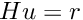where H is a matrix field. This region should be a boundary. The Dirichlet condition is prescribed with a multiplier variable multname which should be first declared as a multiplier variable on the mesh region in the model. dataname is the right hand side of the Dirichlet condition. It could be constant or described on a fem; scalar or vector valued, depending on the variable on which the Dirichlet condition is prescribed. ‘Hname’ is the data corresponding to the matrix field H. It has to be a constant matrix or described on a scalar fem. Return the brick index in the model.

Definition at line 4785 of file getfem_models.cc.

 size_type getfem::add_generalized_Dirichlet_condition_with_multipliers ( model & md, const mesh_im & mim, const std::string & varname, const mesh_fem & mf_mult, size_type region, const std::string & dataname, const std::string & Hname )

Same function as the preceeding one but the multipliers variable will be declared to the brick by the function.

mf_mult is the finite element method on which the multiplier will be build (it will be restricted to the mesh region region and eventually some conflicting dofs with some other multiplier variables will be suppressed).

Definition at line 4800 of file getfem_models.cc.

 size_type getfem::add_generalized_Dirichlet_condition_with_multipliers ( model & md, const mesh_im & mim, const std::string & varname, dim_type degree, size_type region, const std::string & dataname, const std::string & Hname )

Same function as the preceeding one but the mf_mult parameter is replaced by degree.

The multiplier will be described on a standard finite element method of the corresponding degree.

Definition at line 4810 of file getfem_models.cc.

 size_type getfem::add_generalized_Dirichlet_condition_with_penalization ( model & md, const mesh_im & mim, const std::string & varname, scalar_type penalization_coeff, size_type region, const std::string & dataname, const std::string & Hname, const mesh_fem * mf_mult = 0 )

Add a Dirichlet condition on the variable varname and the mesh region region.

This version is for vector field. It prescribes a conditionwhere H is a matrix field. This region should be a boundary. This region should be a boundary. The Dirichlet condition is prescribed with penalization. The penalization coefficient is intially penalization_coeff and will be added to the data of the model. dataname is the right hand side of the Dirichlet condition. It could be constant or described on a fem; scalar or vector valued, depending on the variable on which the Dirichlet condition is prescribed. ‘Hname’ is the data corresponding to the matrix field H. It has to be a constant matrix or described on a scalar fem. mf_mult is an optional parameter which allows to weaken the Dirichlet condition specifying a multiplier space. Return the brick index in the model.

Definition at line 4821 of file getfem_models.cc.

 size_type getfem::add_generalized_Dirichlet_condition_with_Nitsche_method ( model & md, const mesh_im & mim, const std::string & varname, const std::string & Neumannterm, const std::string & datagamma0, size_type region, scalar_type theta, const std::string & datag, const std::string & dataH )

Add a Dirichlet condition on the variable varname and the mesh region region.

This region should be a boundary. This version is for vector field. It prescribes a conditionwhere H is a matrix field. Neumannterm is the expression of the Neumann term (obtained by the Green formula) described as an expression of the high-level generic assembly language. of the high-level generic assembly language. This term can be obtained with md.Neumann_term(varname, region) once all volumic bricks have been added to the model. The Dirichlet condition is prescribed with Nitsche's method. datag is the optional right hand side of the Dirichlet condition. datagamma0 is the Nitsche's method parameter. theta is a scalar value which can be positive or negative. theta = 1 corresponds to the standard symmetric method which is conditionnaly coercive for gamma0 small. theta = -1 corresponds to the skew-symmetric method which is inconditionnaly coercive. theta = 0 is the simplest method for which the second derivative of the Neumann term is not necessary even for nonlinear problems. Return the brick index in the model.

Definition at line 5107 of file getfem_models.cc.

 size_type getfem::add_Helmholtz_brick ( model & md, const mesh_im & mim, const std::string & varname, const std::string & dataexpr, size_type region = size_type(-1) )

Add a Helmoltz brick to the model.

This corresponds to the scalar equation (, with). The weak formulation is (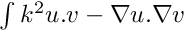)

dataexpr should contain the wave number $k$. It can be real or complex.

Definition at line 5516 of file getfem_models.cc.

 size_type getfem::add_Fourier_Robin_brick ( model & md, const mesh_im & mim, const std::string & varname, const std::string & dataexpr, size_type region )

Add a Fourier-Robin brick to the model.

This correspond to the weak term (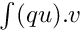) on a boundary. It is used to represent a Fourier-Robin boundary condition.

dataexpr is the parameter $q$ which should be a (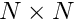) matrix term, where $N$ is the dimension of the variable varname. It can be an arbitrary valid expression of the high-level generic assembly language (except for the complex version for which it should be a data of the model). Note that an additional right hand side can be added with a source term brick.

Definition at line 5637 of file getfem_models.cc.

## ◆ set_private_data_matrix()

template<typename MAT >
 void getfem::set_private_data_matrix ( model & md, size_type indbrick, const MAT & B )

For some specific bricks having an internal sparse matrix (explicit bricks: 'constraint brick' and 'explicit matrix brick'), set this matrix.

@

Definition at line 2267 of file getfem_models.h.

template<typename MAT , typename VECT >
 size_type getfem::add_constraint_with_penalization ( model & md, const std::string & varname, scalar_type penalisation_coeff, const MAT & B, const VECT & L )

Add an additional explicit penalized constraint on the variable varname.

The constraint is $BU=L$ with B being a rectangular sparse matrix. Be aware that B should not contain a plain row, otherwise the whole tangent matrix will be plain. It is possible to change the constraint at any time with the methods set_private_matrix and set_private_rhs. The method change_penalization_coeff can also be used.

Definition at line 2283 of file getfem_models.h.

template<typename MAT , typename VECT >
 size_type getfem::add_constraint_with_multipliers ( model & md, const std::string & varname, const std::string & multname, const MAT & B, const VECT & L )

Add an additional explicit constraint on the variable varname thank to a multiplier multname peviously added to the model (should be a fixed size variable).

The constraint is $BU=L$ with B being a rectangular sparse matrix. It is possible to change the constraint at any time with the methods set_private_matrix and set_private_rhs.

Definition at line 2303 of file getfem_models.h.

template<typename MAT >
 size_type getfem::add_explicit_matrix ( model & md, const std::string & varname1, const std::string & varname2, const MAT & B, bool issymmetric = false, bool iscoercive = false )

Add a brick reprenting an explicit matrix to be added to the tangent linear system relatively to the variables 'varname1' and 'varname2'.

The given matrix should have as many rows as the dimension of 'varname1' and as many columns as the dimension of 'varname2'. If the two variables are different and if ‘issymmetric’ is set to true then the transpose of the matrix is also added to the tangent system (default is false). set iscoercive to true if the term does not affect the coercivity of the tangent system (default is false). The matrix can be changed by the command set_private_matrix.

Definition at line 2337 of file getfem_models.h.

template<typename VECT >
 size_type getfem::add_explicit_rhs ( model & md, const std::string & varname, const VECT & L )

Add a brick representing an explicit right hand side to be added to the right hand side of the tangent linear system relatively to the variable 'varname'.

The given rhs should have the same size than the dimension of 'varname'. The rhs can be changed by the command set_private_rhs.

Definition at line 2354 of file getfem_models.h.

 size_type getfem::add_isotropic_linearized_elasticity_brick ( model & md, const mesh_im & mim, const std::string & varname, const std::string & dataname_lambda, const std::string & dataname_mu, size_type region = size_type(-1), const std::string & dataname_preconstraint = std::string() )

Linear elasticity brick ().

for isotropic material. Parametrized by the Lamé coefficients lambda and mu.

Definition at line 6106 of file getfem_models.cc.

 size_type getfem::add_isotropic_linearized_elasticity_pstrain_brick ( model & md, const mesh_im & mim, const std::string & varname, const std::string & data_E, const std::string & data_nu, size_type region )

Linear elasticity brick ().

for isotropic material. Parametrized by Young modulus and Poisson ratio For two-dimensional problems, corresponds to the plane strain approximation (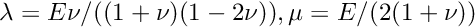). Corresponds to the standard model for three-dimensional problems.

Definition at line 6142 of file getfem_models.cc.

 size_type getfem::add_isotropic_linearized_elasticity_pstress_brick ( model & md, const mesh_im & mim, const std::string & varname, const std::string & data_E, const std::string & data_nu, size_type region )

Linear elasticity brick ().

for isotropic material. Parametrized by Young modulus and Poisson ratio. For two-dimensional problems, corresponds to the plane stress approximation (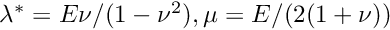). Corresponds to the standard model for three-dimensional problems.

Definition at line 6171 of file getfem_models.cc.

## ◆ compute_isotropic_linearized_Von_Mises_pstrain()

 void getfem::compute_isotropic_linearized_Von_Mises_pstrain ( model & md, const std::string & varname, const std::string & data_E, const std::string & data_nu, const mesh_fem & mf_vm, model_real_plain_vector & VM )

Compute the Von-Mises stress of a displacement field for isotropic linearized elasticity in 3D or in 2D with plane strain assumption.

Parametrized by Young modulus and Poisson ratio.

Definition at line 6256 of file getfem_models.cc.

## ◆ compute_isotropic_linearized_Von_Mises_pstress()

 void getfem::compute_isotropic_linearized_Von_Mises_pstress ( model & md, const std::string & varname, const std::string & data_E, const std::string & data_nu, const mesh_fem & mf_vm, model_real_plain_vector & VM )

Compute the Von-Mises stress of a displacement field for isotropic linearized elasticity in 3D or in 2D with plane stress assumption.

Parametrized by Young modulus and Poisson ratio.

Definition at line 6271 of file getfem_models.cc.

 size_type getfem::add_linear_incompressibility ( model & md, const mesh_im & mim, const std::string & varname, const std::string & multname_pressure, size_type region = size_type(-1), const std::string & dataexpr_penal_coeff = std::string() )

Mixed linear incompressibility condition brick.

Update the tangent matrix with a pressure term: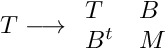withand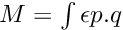(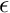is an optional penalization coefficient).

Be aware that an inf-sup condition between the finite element method describing the rpressure and the primal variable has to be satisfied.

For nearly incompressible elasticity,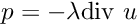Definition at line 6377 of file getfem_models.cc.

 size_type getfem::add_mass_brick ( model & md, const mesh_im & mim, const std::string & varname, const std::string & dataexpr_rho = std::string(), size_type region = size_type(-1) )

Mass brick ().

Add a mass matix on a variable (eventually with a specified region). If the parameter $\rho$ is omitted it is assumed to be equal to 1.

Definition at line 6526 of file getfem_models.cc.

 size_type getfem::add_lumped_mass_for_first_order_brick ( model & md, const mesh_im & mim, const std::string & varname, const std::string & dataexpr_rho = std::string(), size_type region = size_type(-1) )

Lumped mass brick for first order.

Add a lumped mass matix for first order on a variable (eventually with a specified region). If the parameter $\rho$ is omitted it is assumed to be equal to 1.

Definition at line 6616 of file getfem_models.cc.

 size_type getfem::add_basic_d_on_dt_brick ( model & md, const mesh_im & mim, const std::string & varname, const std::string & dataname_dt, const std::string & dataname_rho = std::string(), size_type region = size_type(-1) )

Basic d/dt brick ().

Add the standard discretization of a first order time derivative. The parameter $rho$ is the density which could be omitted (the defaul value is 1). This brick should be used in addition to a time dispatcher for the other terms.

Definition at line 6781 of file getfem_models.cc.

 size_type getfem::add_basic_d2_on_dt2_brick ( model & md, const mesh_im & mim, const std::string & varnameU, const std::string & datanameV, const std::string & dataname_dt, const std::string & dataname_alpha, const std::string & dataname_rho = std::string(), size_type region = size_type(-1) )

Basic d2/dt2 brick ().

Add the standard discretization of a second order time derivative. The parameter $rho$ is the density which could be omitted (the defaul value is 1). This brick should be used in addition to a time dispatcher for the other terms. The time derivative $v$ of the variable $u$ is preferably computed as a post-traitement which depends on each scheme.

Definition at line 6956 of file getfem_models.cc.

 size_type getfem::add_nonlinear_elasticity_brick ( model & md, const mesh_im & mim, const std::string & varname, const phyperelastic_law & AHL, const std::string & dataname, size_type region = size_type(-1) )

Add a nonlinear (large strain) elasticity term to the model with respect to the variable varname (deprecated brick, use add_finite_strain_elaticity instead).

Note that the constitutive law is described by AHL which should not be freed while the model is used. dataname described the parameters of the constitutive laws. It could be a vector of value of length the number of parameter of the constitutive law or a vector field described on a finite element method.

Definition at line 1020 of file getfem_nonlinear_elasticity.cc.

 size_type getfem::add_finite_strain_elasticity_brick ( model & md, const mesh_im & mim, const std::string & lawname, const std::string & varname, const std::string & params, size_type region = size_type(-1) )

Add a finite strain elasticity brick to the model with respect to the variable varname (the displacement).

For 2D meshes, switch automatically to plane strain elasticity. High-level generic assembly version.

Definition at line 2300 of file getfem_nonlinear_elasticity.cc.

 size_type getfem::add_finite_strain_incompressibility_brick ( model & md, const mesh_im & mim, const std::string & varname, const std::string & multname, size_type region = size_type(-1) )

Add a finite strain incompressibility term (for large strain elasticity) to the model with respect to the variable varname (the displacement) and multname (the pressure).

High-level generic assembly version.

Definition at line 2326 of file getfem_nonlinear_elasticity.cc.

## ◆ select_dofs_from_im()

 dal::bit_vector getfem::select_dofs_from_im ( const mesh_fem & mf, const mesh_im & mim, unsigned P = unsigned(-1) )

Return a selection of dof who contribute significantly to the mass-matrix that would be computed with mf and the integration method mim.

P represents the dimension on what the integration method operates (default mf.linked_mesh().dim()).

An example of use can be found in the contrib/xfem_contact/ directory.

A more efficient algorithm is now present in gmm_range_basis.h

Definition at line 116 of file getfem_partial_mesh_fem.cc.

 size_type getfem::add_small_strain_elastoplasticity_brick ( model & md, const mesh_im & mim, std::string lawname, plasticity_unknowns_type unknowns_type, const std::vector< std::string > & varnames, const std::vector< std::string > & params, size_type region = size_type(-1) )

Adds a small strain plasticity term to the model md.

This is the main GetFEM brick for small strain plasticity. lawname is the name of an implemented plastic law, unknowns_type indicates the choice between a discretization where the plastic multiplier is an unknown of the problem or (return mapping approach) just a data of the model stored for the next iteration. Remember that in both cases, a multiplier is stored anyway. varnames is a set of variable and data names with length which may depend on the plastic law (at least the displacement, the plastic multiplier and the plastic strain). params is a list of expressions for the parameters (at least elastic coefficients and the yield stress). These expressions can be some data names (or even variable names) of the model but can also be any scalar valid expression of the high level assembly language (such as "1/2", "2+sin(X)", "1+Norm(v)" ...). The last two parameters optionally provided in params are the theta parameter of the theta-scheme (generalized trapezoidal rule) used for the plastic strain integration and the time-stepdt. The default value for theta if omitted is 1, which corresponds to the classical Backward Euler scheme which is first order consistent. theta=1/2 corresponds to the Crank-Nicolson scheme (trapezoidal rule) which is second order consistent. Any value between 1/2 and 1 should be a valid value. The default value of dt is 'timestep' which simply indicates the time step defined in the model (by md.set_time_step(dt)). Alternatively it can be any expression (data name, constant value ...). The time step can be altered from one iteration to the next one. region is a mesh region.

The available plasticity laws are:

• "Prandtl Reuss" (or "isotropic perfect plasticity"). Isotropic elasto-plasticity with no hardening. The variables are the displacement, the plastic multiplier and the plastic strain. The displacement should be a variable and have a corresponding data having the same name preceded by "Previous_" corresponding to the displacement at the previous time step (typically "u" and "Previous_u"). The plastic multiplier should also have two versions (typically "xi" and "Previous_xi") the first one being defined as data if unknowns_type = DISPLACEMENT_ONLY or as a variable if unknowns_type = DISPLACEMENT_AND_PLASTIC_MULTIPLIER. The plastic strain should represent a n x n data tensor field stored on mesh_fem or (preferably) on an im_data (corresponding to mim). The data are the first Lame coefficient, the second one (shear modulus) and the uniaxial yield stress. IMPORTANT: Note that this law implements the 3D expressions. If it is used in 2D, the expressions are just transposed to the 2D. For the plane strain approximation, see below.
• "plane strain Prandtl Reuss" (or "plane strain isotropic perfect plasticity") The same law as the previous one but adapted to the plane strain approximation. Can only be used in 2D.
• "Prandtl Reuss linear hardening" (or "isotropic plasticity linear hardening"). Isotropic elasto-plasticity with linear isotropic and kinematic hardening. An additional variable compared to "Prandtl Reuss" law: the accumulated plastic strain. Similarly to the plastic strain, it is only stored at the end of the time step, so a simple data is required (preferably on an im_data). Two additional parameters: the kinematic hardening modulus and the isotropic one. 3D expressions only.
• "plane strain Prandtl Reuss linear hardening" (or "plane strain isotropic plasticity linear hardening"). The same law as the previous one but adapted to the plane strain approximation. Can only be used in 2D.

See GetFEM user documentation for further explanations on the discretization of the plastic flow and on the implemented plastic laws. See also GetFEM user documentation on time integration strategy (integration of transient problems).

IMPORTANT : remember that small_strain_elastoplasticity_next_iter has to be called at the end of each time step, before the next one (and before any post-treatment : this sets the value of the plastic strain and plastic multiplier).

Definition at line 1270 of file getfem_plasticity.cc.

## ◆ small_strain_elastoplasticity_next_iter()

 void getfem::small_strain_elastoplasticity_next_iter ( model & md, const mesh_im & mim, std::string lawname, plasticity_unknowns_type unknowns_type, const std::vector< std::string > & varnames, const std::vector< std::string > & params, size_type region = size_type(-1) )

Function that allows to pass from a time step to another for the small strain plastic brick.

The parameters have to be exactly the same as the ones of the add_small_strain_elastoplasticity_brick, so see the documentation of this function for any explanations. Basically, this brick computes the plastic strain and the plastic multiplier and stores them for the next step. Additionaly, it copies the computed displacement to the data that stores the displacement of the previous time step (typically "u" to "Previous_u"). It has to be called before any use of compute_small_strain_elastoplasticity_Von_Mises.

Definition at line 1301 of file getfem_plasticity.cc.

## ◆ compute_small_strain_elastoplasticity_Von_Mises()

 void getfem::compute_small_strain_elastoplasticity_Von_Mises ( model & md, const mesh_im & mim, std::string lawname, plasticity_unknowns_type unknowns_type, const std::vector< std::string > & varnames, const std::vector< std::string > & params, const mesh_fem & mf_vm, model_real_plain_vector & VM, size_type region = size_type(-1) )

This function computes the Von Mises stress field with respect to a small strain elastoplasticity term, approximated on mf_vm, and stores the result into VM.

All other parameters have to be exactly the same as for add_small_strain_elastoplasticity_brick. Remember that small_strain_elastoplasticity_next_iter has to be called before any call of this function.

Definition at line 1373 of file getfem_plasticity.cc.

## ◆ ga_define_linear_hardening_function()

 void getfem::ga_define_linear_hardening_function ( const std::string & name, scalar_type sigma_y0, scalar_type H, bool frobenius = true )

Add a linear function with the name specified by name to represent linear isotropoc hardening in plasticity with initial yield limit sigma_y0 and hardening modulus H.

A true value of the frobenius argument will express the hardening function in terms of Frobenius norms both for the strain input and the stress output, instead of the corresponding Von-Mises quantities.

Definition at line 1420 of file getfem_plasticity.cc.

## ◆ ga_define_Ramberg_Osgood_hardening_function() [1/2]

 void getfem::ga_define_Ramberg_Osgood_hardening_function ( const std::string & name, scalar_type sigma_ref, scalar_type eps_ref, scalar_type n, bool frobenius = false )

Add a Ramberg-Osgood hardening function with the name specified by name, for reference stress and strain given by sigma_ref and eps_ref respectively and for a hardening exponent n.

A true value of the frobenius argument will express the hardening function in terms of Frobenius norms both for the strain input and the stress output, instead of the corresponding Von-Mises quantities.

Definition at line 1433 of file getfem_plasticity.cc.

## ◆ ga_define_Ramberg_Osgood_hardening_function() [2/2]

 void getfem::ga_define_Ramberg_Osgood_hardening_function ( const std::string & name, scalar_type sigma_ref, scalar_type E, scalar_type alpha, scalar_type n, bool frobenius = false )
inline

Add a Ramberg-Osgood hardening function with the name specified by name, for reference stress sigma_ref, Young's modulus E, offset parameter alpha and hardening parameter n.

A true value of the frobenius argument will express the hardening function in terms of Frobenius norms both for the strain input and the stress output, instead of the corresponding Von-Mises quantities.

Definition at line 386 of file getfem_plasticity.h.

 size_type getfem::add_finite_strain_elastoplasticity_brick ( model & md, const mesh_im & mim, std::string lawname, plasticity_unknowns_type unknowns_type, const std::vector< std::string > & varnames, const std::vector< std::string > & params, size_type region = size_type(-1) )

Add a finite strain elastoplasticity brick to the model.

For the moment there is only one supported law defined through lawname as "Simo_Miehe". This law supports to possibilities of unknown variables to solve for defined by means of unknowns_type set to either DISPLACEMENT_AND_PLASTIC_MULTIPLIER or DISPLACEMENT_AND_PLASTIC_MULTIPLIER_AND_PRESSURE The "Simo_Miehe" law expects as varnames a vector of the following names that have to be defined as variables in the model:

• the displacement variable which has to be defined as an unknown,
• the plastic multiplier which has also defined as an unknown,
• optionally the pressure variable for a mixed displacement-pressure formulation for DISPLACEMENT_AND_PLASTIC_MULTIPLIER_AND_PRESSURE as unknowns_type,
• the name of a (scalar) fem_data or im_data field that holds the plastic strain at the previous time step, and
• the name of a fem_data or im_data field that holds all non-repeated components of the inverse of the plastic right Cauchy-Green tensor at the previous time step (it has to be a 4 element vector for plane strain 2D problems and a 6 element vector for 3D problems). The "Simo_Miehe" law also expects as params a vector of the following three parameters:
• an expression for the initial bulk modulus K,
• an expression for the initial shear modulus G,
• the name of a user predefined function that decribes the yield limit as a function of the hardening variable (both the yield limit and the hardening variable values are assumed to be Frobenius norms of appropriate stress and strain tensors, respectively),

## ◆ finite_strain_elastoplasticity_next_iter()

 void getfem::finite_strain_elastoplasticity_next_iter ( model & md, const mesh_im & mim, std::string lawname, plasticity_unknowns_type unknowns_type, const std::vector< std::string > & varnames, const std::vector< std::string > & params, size_type region = size_type(-1) )

This function permits to update the state variables for a finite strain elastoplasticity brick, based on the current displacements and plastic multiplier fields (optionally also the the pressure field in the case of a mixed displacement-pressure formulation).

The parameters have to be exactly the same as the ones of the add_finite_strain_elastoplasticity_brick, so see the documentation of this function for any explanations. Basically, varnames contains both the names of the input fields as well as the names of the fields to be updated.

## ◆ compute_finite_strain_elastoplasticity_Von_Mises()

 void getfem::compute_finite_strain_elastoplasticity_Von_Mises ( model & md, const mesh_im & mim, std::string lawname, plasticity_unknowns_type unknowns_type, const std::vector< std::string > & varnames, const std::vector< std::string > & params, const mesh_fem & mf_vm, model_real_plain_vector & VM, size_type region = size_type(-1) )

This function computes the Von Mises stress field with respect to a finite strain elastoplasticity term, approximated on mf_vm, and stores the result into VM.

All other parameters have to be exactly the same as for add_finite_strain_elastoplasticity_brick.

 size_type getfem::add_elastoplasticity_brick ( model & md, const mesh_im & mim, const pconstraints_projection & ACP, const std::string & varname, const std::string & previous_dep_name, const std::string & datalambda, const std::string & datamu, const std::string & datathreshold, const std::string & datasigma, size_type region = size_type(-1) )

Add a nonlinear elastoplasticity term to the model for small deformations and an isotropic material, with respect to the variable varname.

Note that the constitutive lawtype of projection to be used is described by ACP which should not be freed while the model is used. datalambda and datamu describe the Lamé coeffcients of the studied material. Could be scalar or vector fields described on a finite element method. datathreshold represents the elasticity threshold of the material. It could be a scalar or a vector field described on the same finite element method as the Lamé coefficients. datasigma represents the stress constraints values supported by the material. It should be a vector field described on a finite element method. previous_dep_name represents the displacement at the previous time step. Moreover, if varname is described onto a K-th order mesh_fem, datasigma has to be described on a mesh_fem of order at least K-1.

## ◆ elastoplasticity_next_iter()

 void getfem::elastoplasticity_next_iter ( model & md, const mesh_im & mim, const std::string & varname, const std::string & previous_dep_name, const pconstraints_projection & ACP, const std::string & datalambda, const std::string & datamu, const std::string & datathreshold, const std::string & datasigma )

This function permits to compute the new stress constraints values supported by the material after a load or an unload.

varname is the main unknown of the problem (the displacement), previous_dep_name represents the displacement at the previous time step, ACP is the type of projection to be used that could only be Von Mises for the moment, datalambda and datamu are the Lamé coefficients of the material, datathreshold is the elasticity threshold of the material, datasigma is the vector which will contains the new computed values.

## ◆ compute_elastoplasticity_Von_Mises_or_Tresca()

 void getfem::compute_elastoplasticity_Von_Mises_or_Tresca ( model & md, const std::string & datasigma, const mesh_fem & mf_vm, model_real_plain_vector & VM, bool tresca )

This function computes on mf_vm the Von Mises or Tresca stress of a field for elastoplasticity and return it into the vector VM.

Note that datasigma should be the vector containing the new stress constraints values, i.e. after a load or an unload of the material. If tresca = 'true', the Tresca stress will be computed, otherwise it will be the Von Mises one.

## ◆ compute_plastic_part()

 void getfem::compute_plastic_part ( model & md, const mesh_im & mim, const mesh_fem & mf_pl, const std::string & varname, const std::string & previous_dep_name, const pconstraints_projection & ACP, const std::string & datalambda, const std::string & datamu, const std::string & datathreshold, const std::string & datasigma, model_real_plain_vector & plast )

This function computes on mf_pl the plastic part, that could appear after a load and an unload, into the vector plast.

Note that datasigma should be the vector containing the new stress constraints values, i.e. after a load or an unload of the material.

## ◆ new_projected_fem()

 pfem getfem::new_projected_fem ( const mesh_fem & mf_source, const mesh_im & mim_target, size_type rg_source_ = size_type(-1), size_type rg_target_ = size_type(-1), dal::bit_vector blocked_dofs = dal::bit_vector(), bool store_val = true )

create a new projected FEM.

Parameters
 mf_source the mesh_fem that will be projected. mim_target the integration method on the final mesh (not the mesh of mf_source!). blocked_dofs list of dof of mf_source which won't be projected. store_val if true, the values/gradients of interpolated base function are cached at each gauss point (eats much memory).

Definition at line 881 of file getfem_projected_fem.cc.

## ◆ regular_unit_mesh()

 void getfem::regular_unit_mesh ( mesh & m, std::vector< size_type > nsubdiv, bgeot::pgeometric_trans pgt, bool noised = false` )

Build a regular mesh of the unit square/cube/, etc.

Parameters
 m the output mesh. pgt the geometric transformation to use. For example, use pgt = geometric_trans_descriptor("GT_PK(2,1"); // to build a mesh of triangles pgt = geometric_trans_descriptor("QK(3,2)"); // to build a mesh of order 2 parallelepiped nsubdiv is the number of cells in each direction. noised if set, will cause the interior nodes to be randomly "shaken".

Definition at line 238 of file getfem_regular_meshes.cc.

## ◆ regular_mesh()

 void getfem::regular_mesh ( mesh & m, const std::string & st )

Build a regular mesh parametrized by the string st.

The format of st is the following std::string st("GT='GT_PK(2,1)'; NSUBDIV=[5,5]; ORG=[0,0]; SIZES=[1,1]; NOISED=0"); where GT is the geometric transformation, NSUBDIV a vector of the number of subdivisions in each coordinate (default value 2), ORG is the origin of the mesh (default value [0,0,...]), SIZES is a vector of the sizes in each direction (default value [1, 1, ...] and if NOISED=1 the nodes of the interior of the mesh are randomly "shaken" (default value NOISED=0). All the parameters are optional but GT.

Parameters
 m the output mesh.

Definition at line 289 of file getfem_regular_meshes.cc.

## ◆ regular_ball_mesh()

 void getfem::regular_ball_mesh ( mesh & m, const std::string & st )

Build a regular mesh on a ball, parametrized by the string st.

The format of st is similar to getfem::regular_mesh.

getfem::import_mesh. All parameters except the geometric transformation GT are optional. Here, parameter NSUBDIV has to be a vector of size 2 that holds the number of subdivisions in the core and the peel sub-regions of the mesh. SIZES is an one element vector that provides the ball radius (default radius is equal to 1). If NOISED=1 the nodes in the interior of the individual sub-regions of the mesh are randomly "shaken" (default value NOISED=0). An additional integer paramater called SYMMETRIES receiving the values 1, 2 or 3 (in three dimensions) permits to respectively request one half, one quarter or one eighth of the ball to be meshed (default value SYMMETRIES=0).
Parameters
 m the output mesh.

Definition at line 352 of file getfem_regular_meshes.cc.

## ◆ regular_ball_shell_mesh()

 void getfem::regular_ball_shell_mesh ( mesh & m, const std::string & st )

Build a regular mesh on a ball shell, parametrized by the string st.

The format of st is similar to getfem::regular_mesh.

getfem::import_mesh. All parameters except the geometric transformation GT are optional. Here, parameter NSUBDIV has to be a vector of size 2 that holds the number of subdivisions in the circumferential and radial direction of the mesh. SIZES is a two element vector that provides the ball radius and shell thickness (default radius is equal to 1 and default thickness is ewual to 0.5). If NOISED=1 the nodes in the interior of the individual sub-regions of the mesh are randomly "shaken" (default value NOISED=0). An additional integer paramater called SYMMETRIES receiving the values 1, 2 or 3 (in three dimensions) permits to respectively request one half, one quarter or one eighth of the ball to be meshed (default value SYMMETRIES=0).
Parameters
 m the output mesh.

Definition at line 505 of file getfem_regular_meshes.cc.

bgeot::geometric_trans_descriptor
pgeometric_trans geometric_trans_descriptor(std::string name)
Get the geometric transformation from its string name.
Definition: bgeot_geometric_trans.cc:1163
getfem::import_mesh_gmsh
void import_mesh_gmsh(const std::string &filename, mesh &m, std::map< std::string, size_type > &region_map, bool remove_last_dimension=true, std::map< size_type, std::set< size_type >> *nodal_map=NULL, bool remove_duplicated_nodes=true)
Import a mesh file in format generated by Gmsh.
Definition: getfem_import.cc:1531
getfem::mesh
Describe a mesh (collection of convexes (elements) and points).
Definition: getfem_mesh.h:95